Anzeige

# GE Lab manual.pdf

26. Mar 2023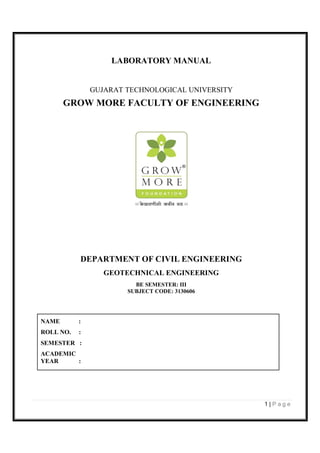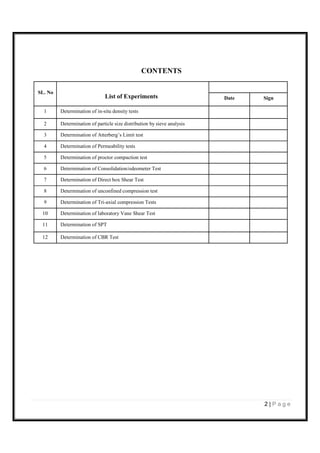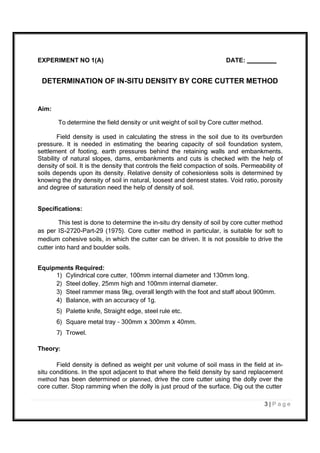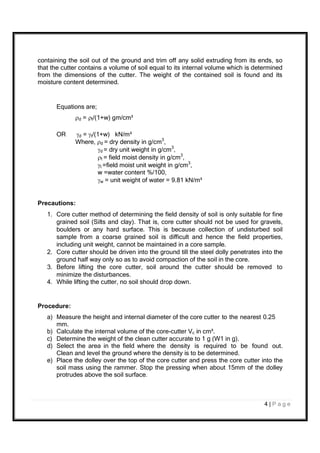Anzeige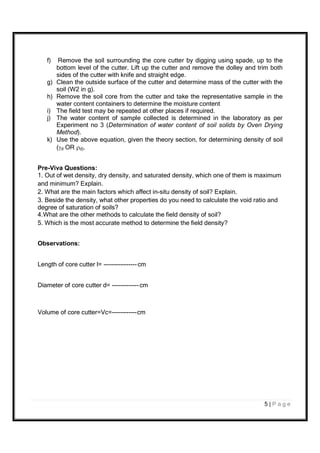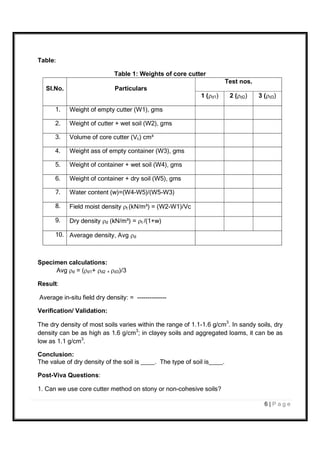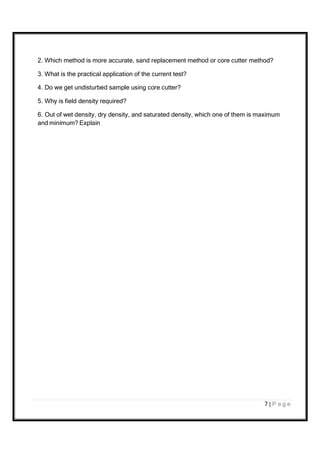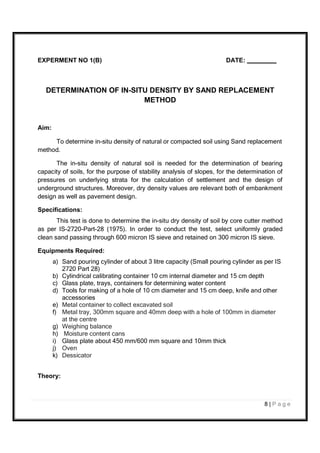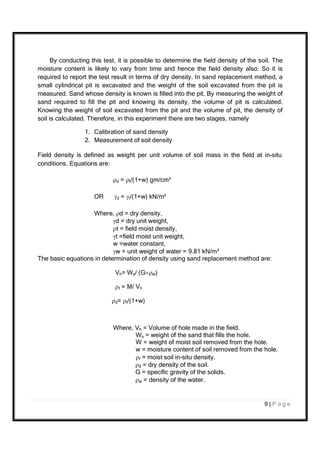Anzeige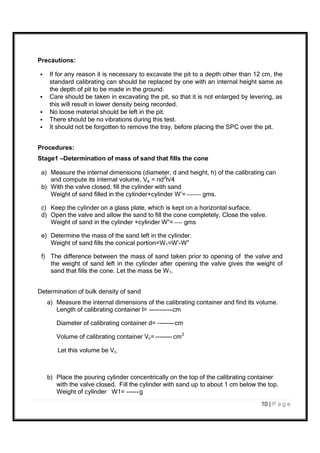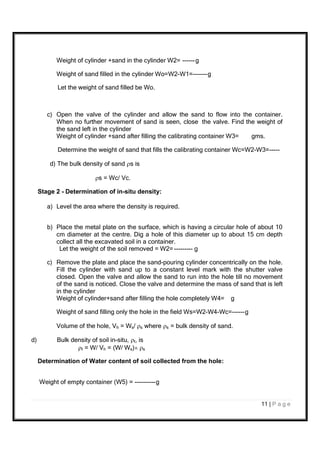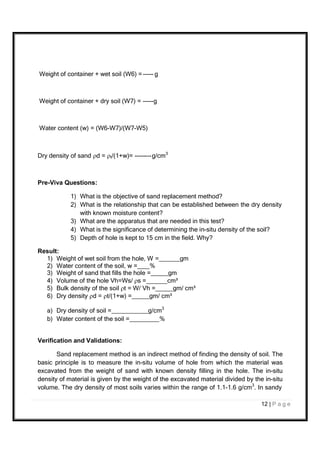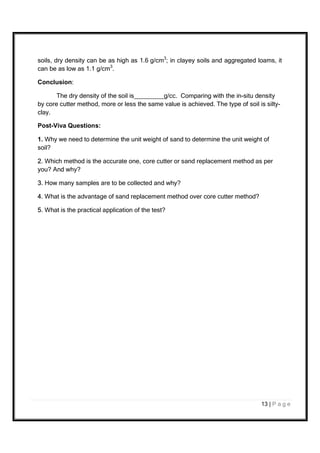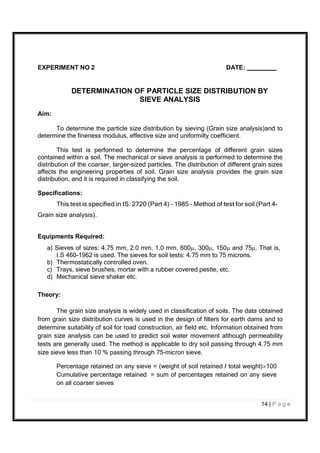Anzeige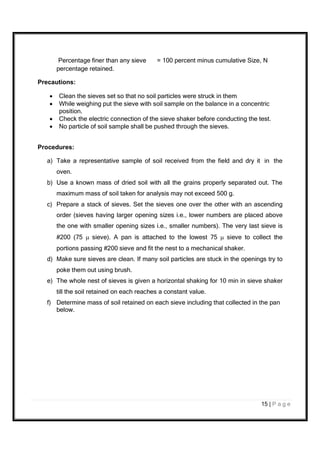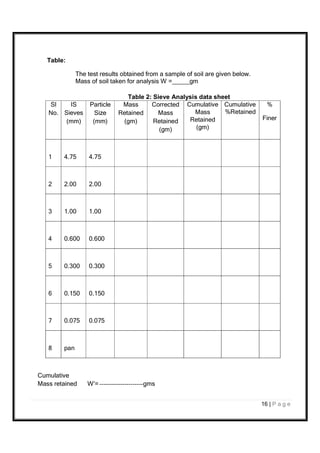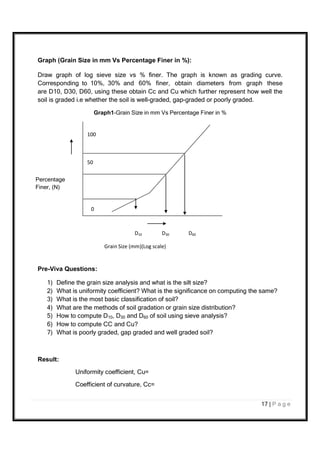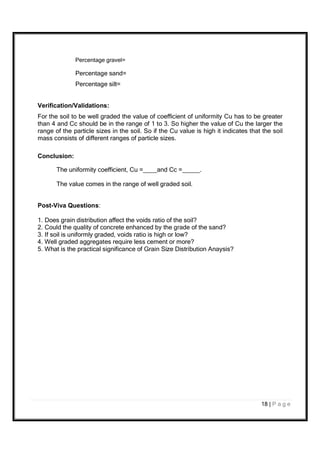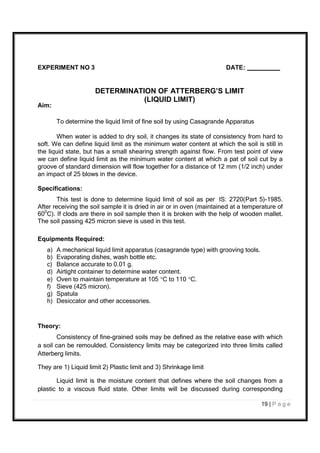Anzeige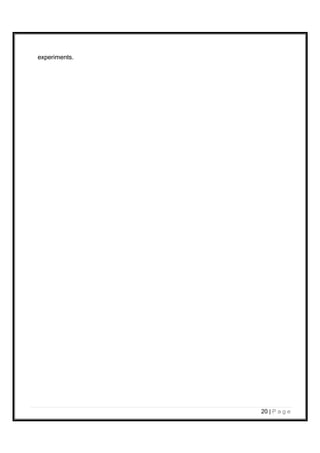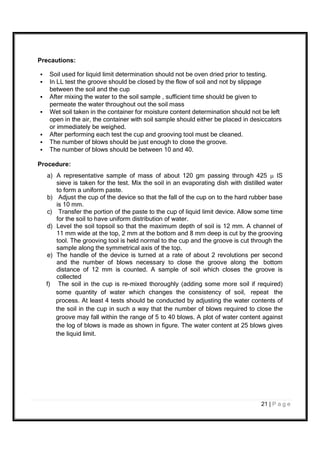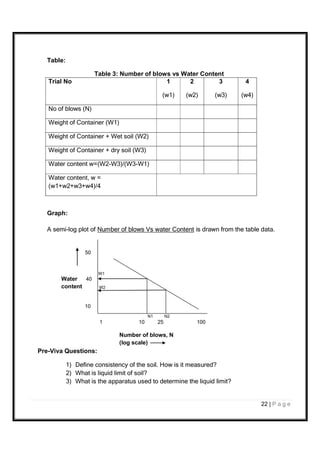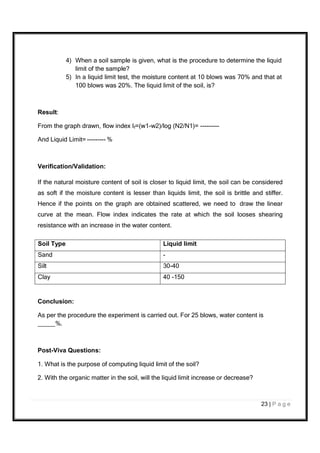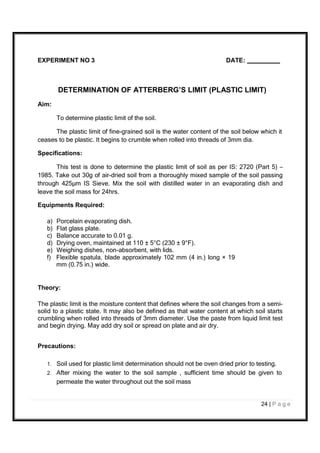Anzeige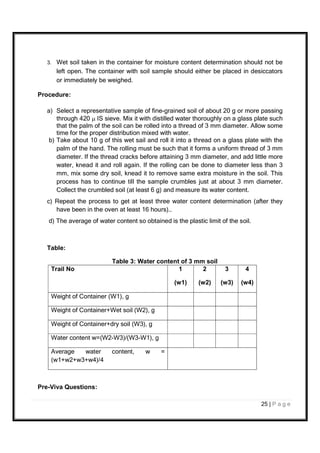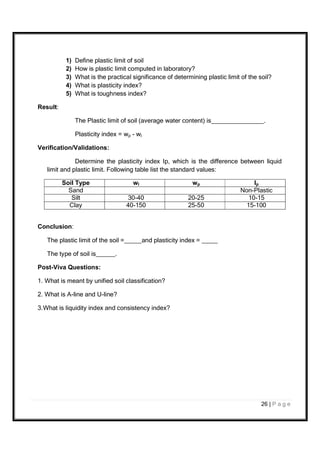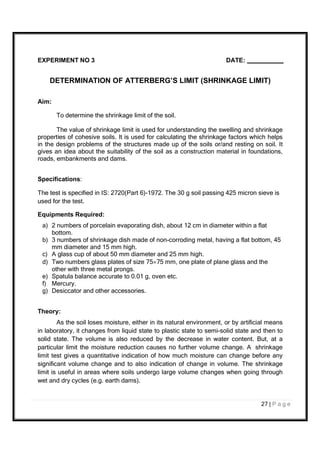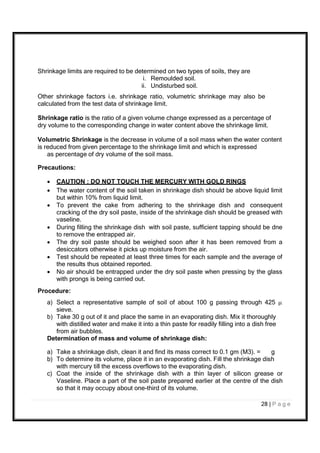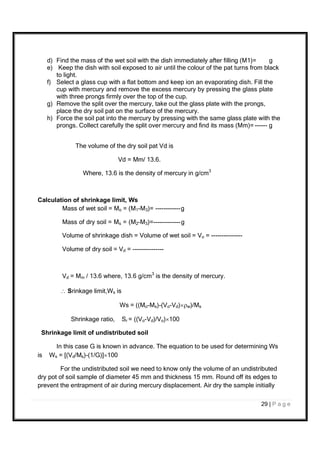Anzeige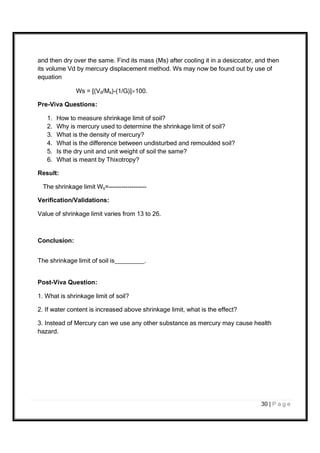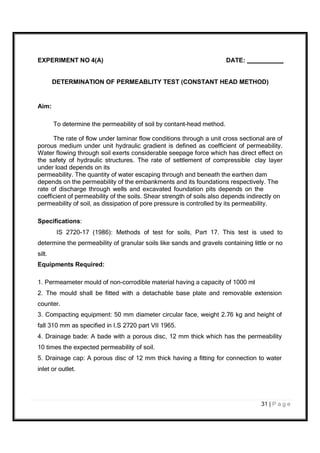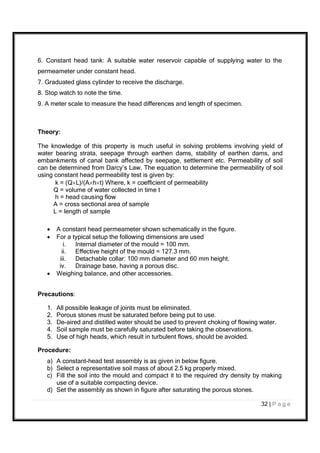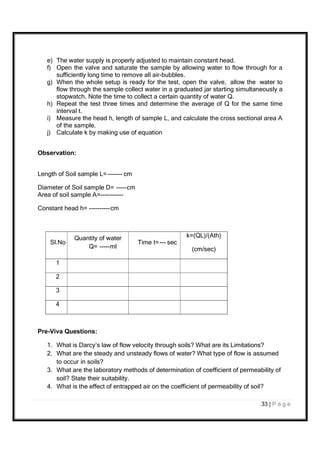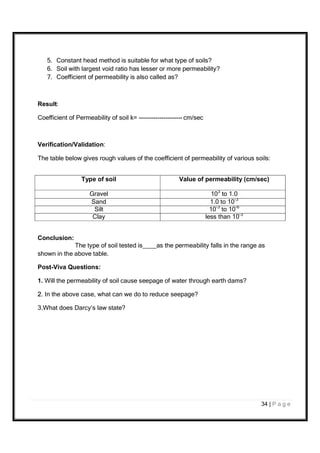Anzeige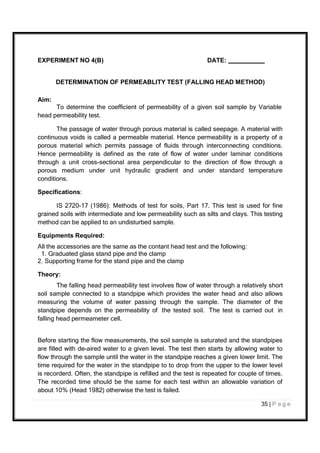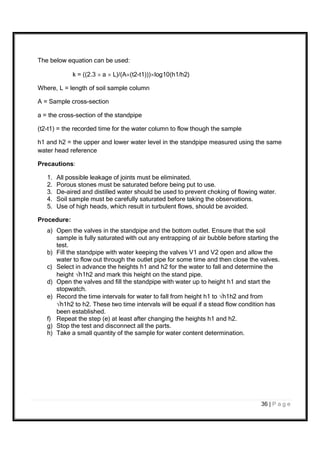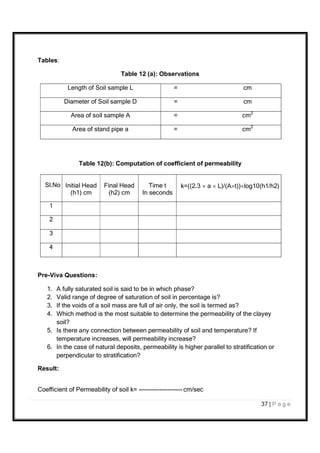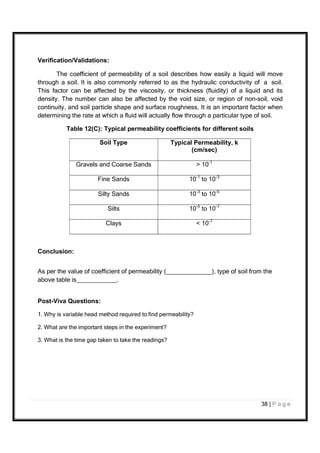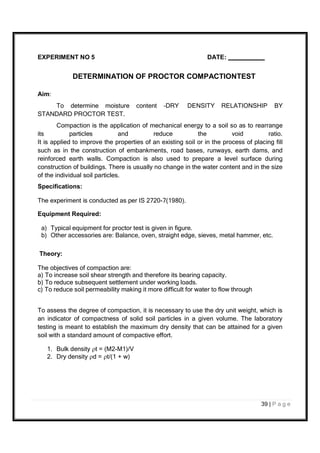Anzeige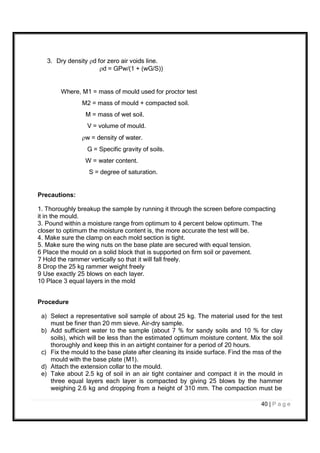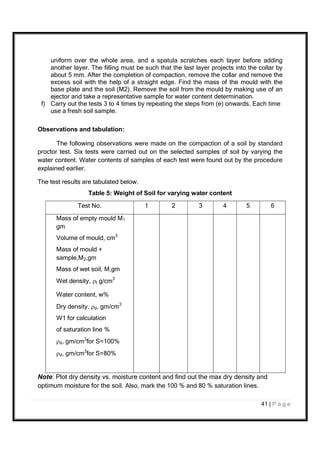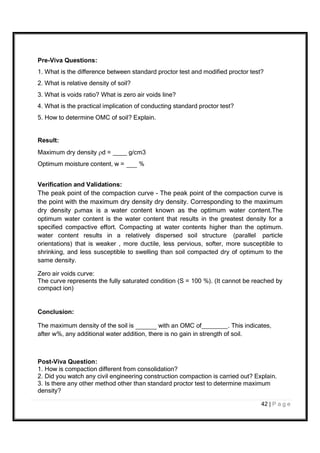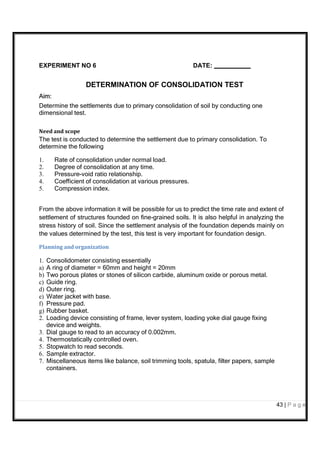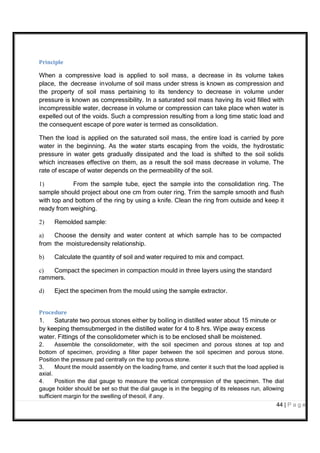Anzeige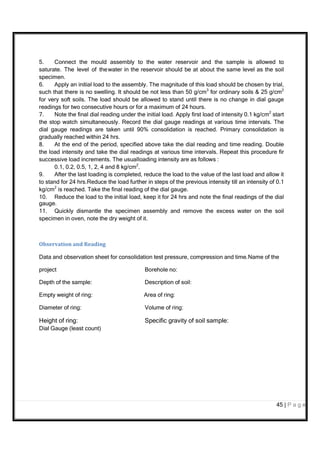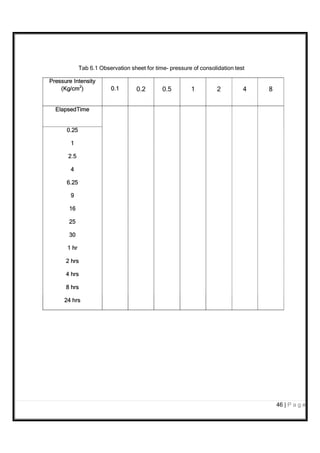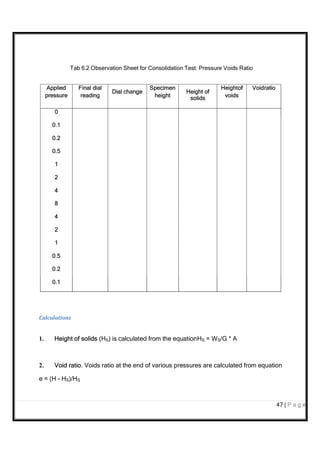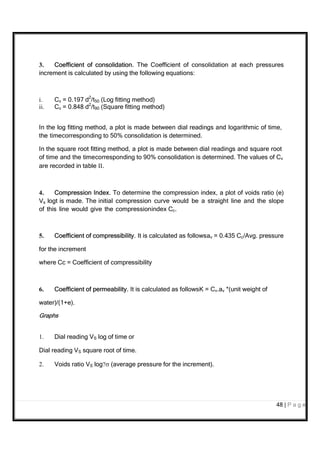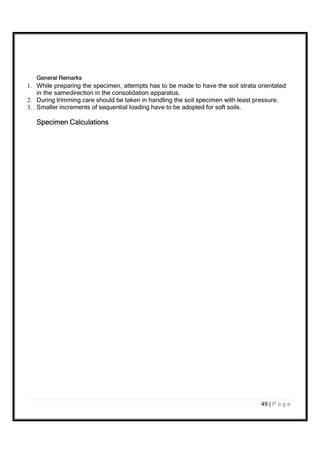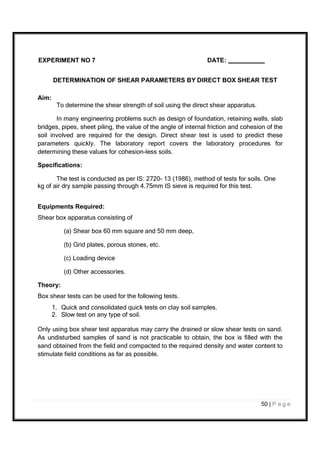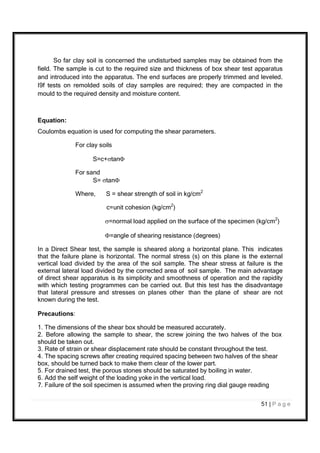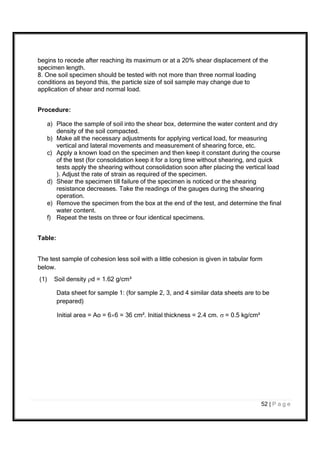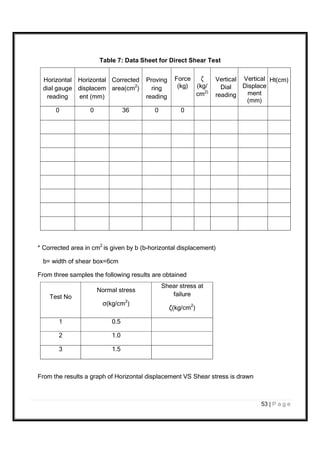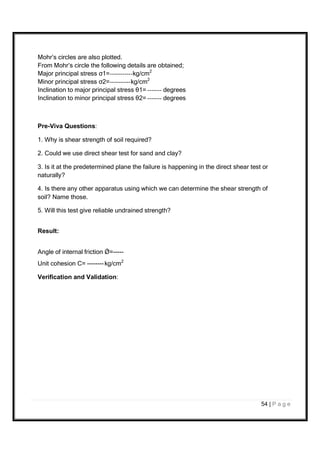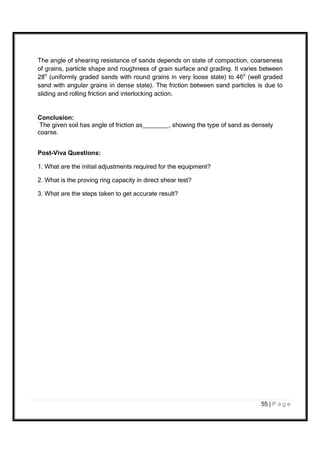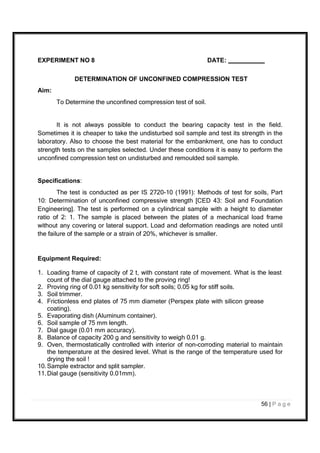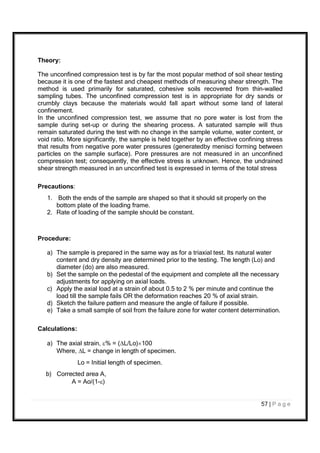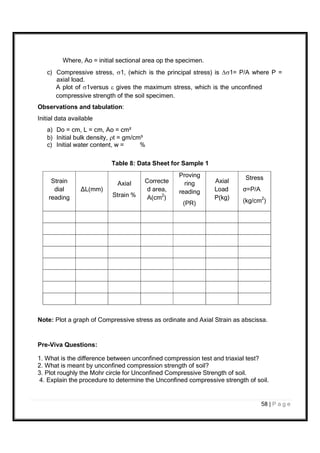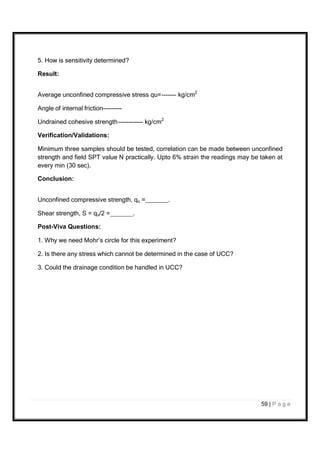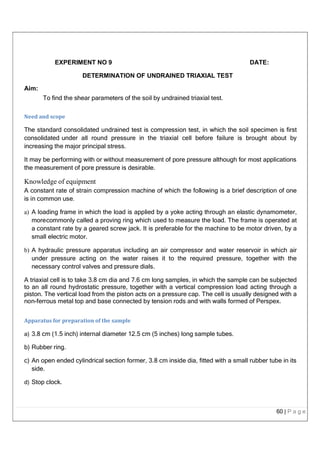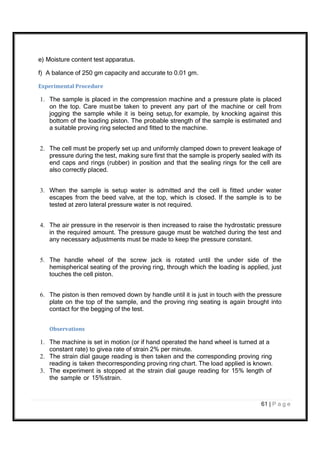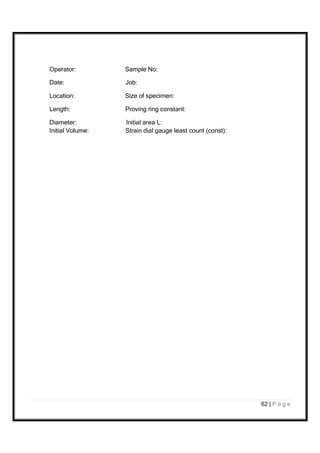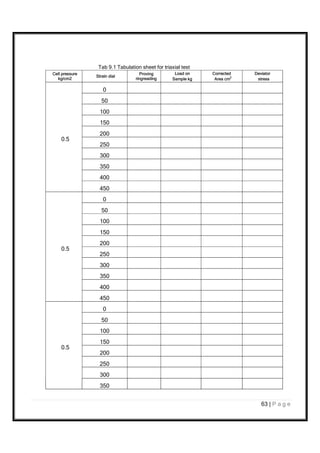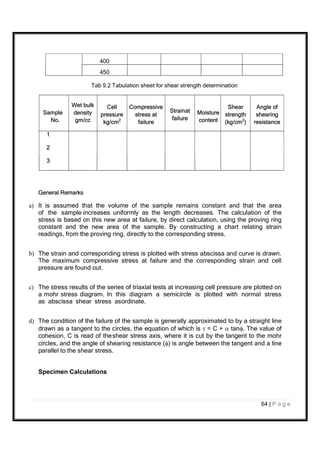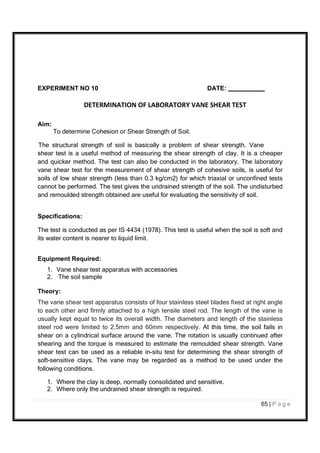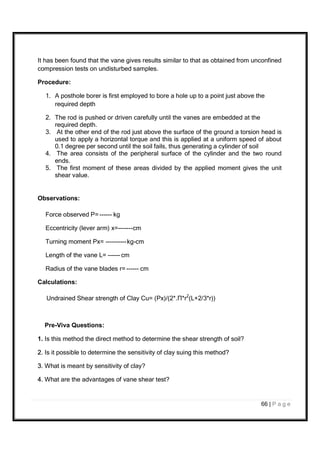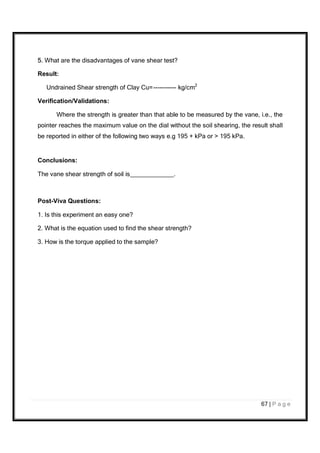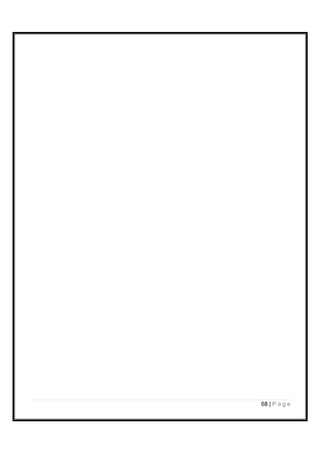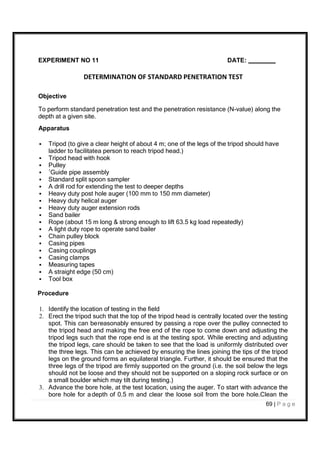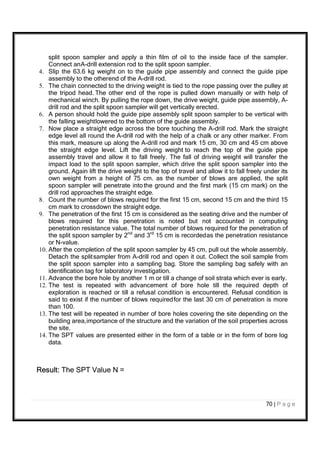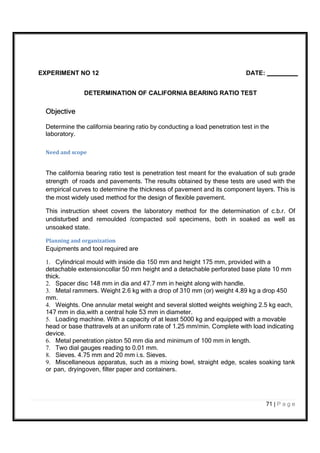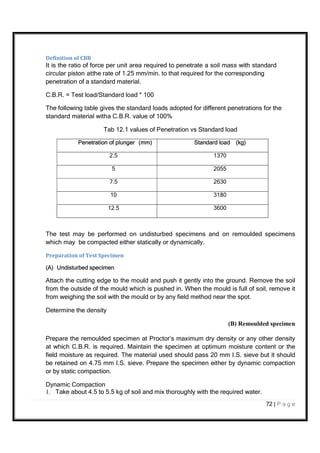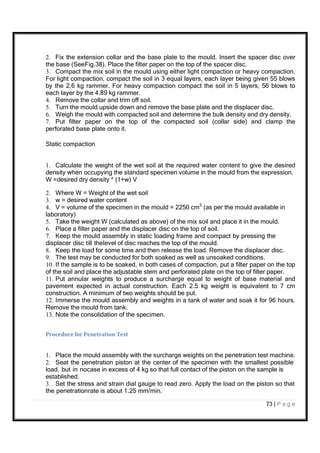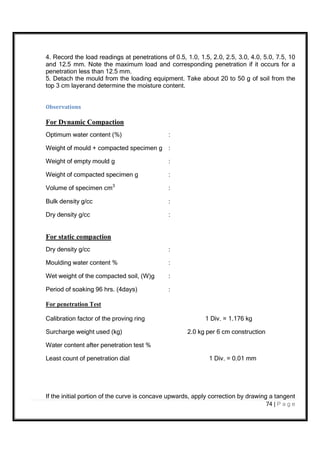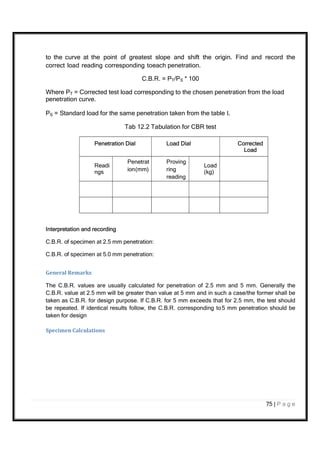Nächste SlideShare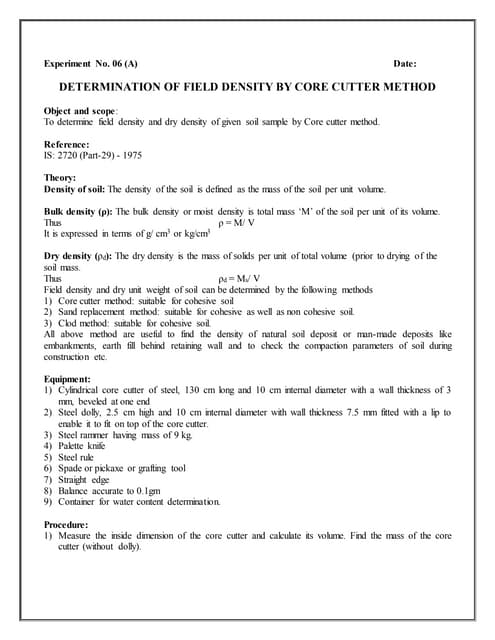Ex 6 a field density by core cutter
1 von 75
Anzeige

### GE Lab manual.pdf

1. 1 | P a g e NAME : ROLL NO. : SEMESTER : ACADEMIC YEAR : LABORATORY MANUAL GUJARAT TECHNOLOGICAL UNIVERSITY GROW MORE FACULTY OF ENGINEERING DEPARTMENT OF CIVIL ENGINEERING GEOTECHNICAL ENGINEERING BE SEMESTER: III SUBJECT CODE: 3130606
2. 2 | P a g e CONTENTS SL. No List of Experiments Date Sign 1 Determination of in-situ density tests 2 Determination of particle size distribution by sieve analysis 3 Determination of Atterberg’s Limit test 4 Determination of Permeability tests 5 Determination of proctor compaction test 6 Determination of Consolidation/odeometer Test 7 Determination of Direct box Shear Test 8 Determination of unconfined compression test 9 Determination of Tri-axial compression Tests 10 Determination of laboratory Vane Shear Test 11 Determination of SPT 12 Determination of CBR Test
3. 3 | P a g e EXPERIMENT NO 1(A) DATE: DETERMINATION OF IN-SITU DENSITY BY CORE CUTTER METHOD Aim: To determine the field density or unit weight of soil by Core cutter method. Field density is used in calculating the stress in the soil due to its overburden pressure. It is needed in estimating the bearing capacity of soil foundation system, settlement of footing, earth pressures behind the retaining walls and embankments. Stability of natural slopes, dams, embankments and cuts is checked with the help of density of soil. It is the density that controls the field compaction of soils. Permeability of soils depends upon its density. Relative density of cohesionless soils is determined by knowing the dry density of soil in natural, loosest and densest states. Void ratio, porosity and degree of saturation need the help of density of soil. Specifications: This test is done to determine the in-situ dry density of soil by core cutter method as per IS-2720-Part-29 (1975). Core cutter method in particular, is suitable for soft to medium cohesive soils, in which the cutter can be driven. It is not possible to drive the cutter into hard and boulder soils. Equipments Required: 1) Cylindrical core cutter, 100mm internal diameter and 130mm long. 2) Steel dolley, 25mm high and 100mm internal diameter. 3) Steel rammer mass 9kg, overall length with the foot and staff about 900mm. 4) Balance, with an accuracy of 1g. 5) Palette knife, Straight edge, steel rule etc. 6) Square metal tray – 300mm x 300mm x 40mm. 7) Trowel. Theory: Field density is defined as weight per unit volume of soil mass in the field at in- situ conditions. In the spot adjacent to that where the field density by sand replacement method has been determined or planned, drive the core cutter using the dolly over the core cutter. Stop ramming when the dolly is just proud of the surface. Dig out the cutter
4. 4 | P a g e containing the soil out of the ground and trim off any solid extruding from its ends, so that the cutter contains a volume of soil equal to its internal volume which is determined from the dimensions of the cutter. The weight of the contained soil is found and its moisture content determined. Equations are; d = t/(1+w) gm/cm³ OR d = t/(1+w) kN/m³ Where, d = dry density in g/cm3 , d = dry unit weight in g/cm3 , t = field moist density in g/cm3 , t =field moist unit weight in g/cm3 , w =water content %/100, w = unit weight of water = 9.81 kN/m³ Precautions: 1. Core cutter method of determining the field density of soil is only suitable for fine grained soil (Silts and clay). That is, core cutter should not be used for gravels, boulders or any hard surface. This is because collection of undisturbed soil sample from a coarse grained soil is difficult and hence the field properties, including unit weight, cannot be maintained in a core sample. 2. Core cutter should be driven into the ground till the steel dolly penetrates into the ground half way only so as to avoid compaction of the soil in the core. 3. Before lifting the core cutter, soil around the cutter should be removed to minimize the disturbances. 4. While lifting the cutter, no soil should drop down. Procedure: a) Measure the height and internal diameter of the core cutter to the nearest 0.25 mm. b) Calculate the internal volume of the core-cutter Vc in cm³. c) Determine the weight of the clean cutter accurate to 1 g (W1 in g). d) Select the area in the field where the density is required to be found out. Clean and level the ground where the density is to be determined. e) Place the dolley over the top of the core cutter and press the core cutter into the soil mass using the rammer. Stop the pressing when about 15mm of the dolley protrudes above the soil surface.
5. 5 | P a g e f) Remove the soil surrounding the core cutter by digging using spade, up to the bottom level of the cutter. Lift up the cutter and remove the dolley and trim both sides of the cutter with knife and straight edge. g) Clean the outside surface of the cutter and determine mass of the cutter with the soil (W2 in g). h) Remove the soil core from the cutter and take the representative sample in the water content containers to determine the moisture content i) The field test may be repeated at other places if required. j) The water content of sample collected is determined in the laboratory as per Experiment no 3 (Determination of water content of soil solids by Oven Drying Method). k) Use the above equation, given the theory section, for determining density of soil (d OR d). Pre-Viva Questions: 1. Out of wet density, dry density, and saturated density, which one of them is maximum and minimum? Explain. 2. What are the main factors which affect in-situ density of soil? Explain. 3. Beside the density, what other properties do you need to calculate the void ratio and degree of saturation of soils? 4.What are the other methods to calculate the field density of soil? 5. Which is the most accurate method to determine the field density? Observations: Length of core cutter l= ----------------cm Diameter of core cutter d= -------------cm Volume of core cutter=Vc=------------cm
6. 6 | P a g e Table: Table 1: Weights of core cutter Sl.No. Particulars Test nos. 1 (d1) 2 (d2) 3 (d3) 1. Weight of empty cutter (W1), gms 2. Weight of cutter + wet soil (W2), gms 3. Volume of core cutter (Vc) cm³ 4. Weight ass of empty container (W3), gms 5. Weight of container + wet soil (W4), gms 6. Weight of container + dry soil (W5), gms 7. Water content (w)=(W4-W5)/(W5-W3) 8. Field moist density t (kN/m³) = (W2-W1)/Vc 9. Dry density d (kN/m³) = t /(1+w) 10. Average density, Avg d Specimen calculations: Avg d = (d1+ d2 + d3)/3 Result: Average in-situ field dry density: = -------------- Verification/ Validation: The dry density of most soils varies within the range of 1.1-1.6 g/cm3 . In sandy soils, dry density can be as high as 1.6 g/cm3 ; in clayey soils and aggregated loams, it can be as low as 1.1 g/cm3 . Conclusion: The value of dry density of the soil is _ . The type of soil is . Post-Viva Questions: 1. Can we use core cutter method on stony or non-cohesive soils?
7. 7 | P a g e 2. Which method is more accurate, sand replacement method or core cutter method? 3. What is the practical application of the current test? 4. Do we get undisturbed sample using core cutter? 5. Why is field density required? 6. Out of wet density, dry density, and saturated density, which one of them is maximum and minimum? Explain
8. 8 | P a g e EXPERMENT NO 1(B) DATE: DETERMINATION OF IN-SITU DENSITY BY SAND REPLACEMENT METHOD Aim: To determine in-situ density of natural or compacted soil using Sand replacement method. The in-situ density of natural soil is needed for the determination of bearing capacity of soils, for the purpose of stability analysis of slopes, for the determination of pressures on underlying strata for the calculation of settlement and the design of underground structures. Moreover, dry density values are relevant both of embankment design as well as pavement design. Specifications: This test is done to determine the in-situ dry density of soil by core cutter method as per IS-2720-Part-28 (1975). In order to conduct the test, select uniformly graded clean sand passing through 600 micron IS sieve and retained on 300 micron IS sieve. Equipments Required: a) Sand pouring cylinder of about 3 litre capacity (Small pouring cylinder as per IS 2720 Part 28) b) Cylindrical calibrating container 10 cm internal diameter and 15 cm depth c) Glass plate, trays, containers for determining water content d) Tools for making of a hole of 10 cm diameter and 15 cm deep, knife and other accessories e) Metal container to collect excavated soil f) Metal tray, 300mm square and 40mm deep with a hole of 100mm in diameter at the centre g) Weighing balance h) Moisture content cans i) Glass plate about 450 mm/600 mm square and 10mm thick j) Oven k) Dessicator Theory:
9. 9 | P a g e By conducting this test, it is possible to determine the field density of the soil. The moisture content is likely to vary from time and hence the field density also. So it is required to report the test result in terms of dry density. In sand replacement method, a small cylindrical pit is excavated and the weight of the soil excavated from the pit is measured. Sand whose density is known is filled into the pit. By measuring the weight of sand required to fill the pit and knowing its density, the volume of pit is calculated. Knowing the weight of soil excavated from the pit and the volume of pit, the density of soil is calculated. Therefore, in this experiment there are two stages, namely 1. Calibration of sand density 2. Measurement of soil density Field density is defined as weight per unit volume of soil mass in the field at in-situ conditions. Equations are: d = t/(1+w) gm/cm³ OR d = t/(1+w) kN/m³ Where, d = dry density, d = dry unit weight, t = field moist density, t =field moist unit weight, w =water constant, w = unit weight of water = 9.81 kN/m³ The basic equations in determination of density using sand replacement method are: Vh= Ws/ (Gw) t = M/ Vh d= t/(1+w) Where, Vh = Volume of hole made in the field. Ws = weight of the sand that fills the hole. W = weight of moist soil removed from the hole. w = moisture content of soil removed from the hole. t = moist soil in-situ density. d = dry density of the soil. G = specific gravity of the solids. w = density of the water.
10. 10 | P a g e Precautions:  If for any reason it is necessary to excavate the pit to a depth other than 12 cm, the standard calibrating can should be replaced by one with an internal height same as the depth of pit to be made in the ground.  Care should be taken in excavating the pit, so that it is not enlarged by levering, as this will result in lower density being recorded.  No loose material should be left in the pit.  There should be no vibrations during this test.  It should not be forgotten to remove the tray, before placing the SPC over the pit. Procedures: Stage1 –Determination of mass of sand that fills the cone a) Measure the internal dimensions (diameter, d and height, h) of the calibrating can and compute its internal volume, Vc = πd2 h/4 b) With the valve closed, fill the cylinder with sand Weight of sand filled in the cylinder+cylinder W’=------- gms. c) Keep the cylinder on a glass plate, which is kept on a horizontal surface. d) Open the valve and allow the sand to fill the cone completely. Close the valve. Weight of sand in the cylinder +cylinder W”=---- gms e) Determine the mass of the sand left in the cylinder. Weight of sand fills the conical portion=W1=W’-W” f) The difference between the mass of sand taken prior to opening of the valve and the weight of sand left in the cylinder after opening the valve gives the weight of sand that fills the cone. Let the mass be W1. Determination of bulk density of sand a) Measure the internal dimensions of the calibrating container and find its volume. Length of calibrating container l= -----------cm Diameter of calibrating container d= --------cm Volume of calibrating container Vc= -------- cm3 Let this volume be Vc. b) Place the pouring cylinder concentrically on the top of the calibrating container with the valve closed. Fill the cylinder with sand up to about 1 cm below the top. Weight of cylinder W1= ------g
11. 11 | P a g e Weight of cylinder +sand in the cylinder W2= ------g Weight of sand filled in the cylinder Wo=W2-W1=-------g Let the weight of sand filled be Wo. c) Open the valve of the cylinder and allow the sand to flow into the container. When no further movement of sand is seen, close the valve. Find the weight of the sand left in the cylinder Weight of cylinder +sand after filling the calibrating container W3= gms. Determine the weight of sand that fills the calibrating container Wc=W2-W3=----- d) The bulk density of sand s is s = Wc/ Vc. Stage 2 - Determination of in-situ density: a) Level the area where the density is required. b) Place the metal plate on the surface, which is having a circular hole of about 10 cm diameter at the centre. Dig a hole of this diameter up to about 15 cm depth collect all the excavated soil in a container. Let the weight of the soil removed = W2=--------- g c) Remove the plate and place the sand-pouring cylinder concentrically on the hole. Fill the cylinder with sand up to a constant level mark with the shutter valve closed. Open the valve and allow the sand to run into the hole till no movement of the sand is noticed. Close the valve and determine the mass of sand that is left in the cylinder Weight of cylinder+sand after filling the hole completely W4= g Weight of sand filling only the hole in the field Ws=W2-W4-Wc=------g Volume of the hole, Vh = Ws/ s where s = bulk density of sand. d) Bulk density of soil in-situ, t, is t = W/ Vh = (W/ Ws) s Determination of Water content of soil collected from the hole: Weight of empty container (W5) = ----------g
12. 12 | P a g e Weight of container + wet soil (W6) =----- g Weight of container + dry soil (W7) = -----g Water content (w) = (W6-W7)/(W7-W5) Dry density of sand d = t/(1+w)= --------g/cm3 Pre-Viva Questions: 1) What is the objective of sand replacement method? 2) What is the relationship that can be established between the dry density with known moisture content? 3) What are the apparatus that are needed in this test? 4) What is the significance of determining the in-situ density of the soil? 5) Depth of hole is kept to 15 cm in the field. Why? Result: 1) Weight of wet soil from the hole, W = gm 2) Water content of the soil, w = % 3) Weight of sand that fills the hole = gm 4) Volume of the hole Vh=Ws/ s = cm³ 5) Bulk density of the soil t = W/ Vh = gm/ cm³ 6) Dry density d = t/(1+w) = gm/ cm³ a) Dry density of soil = g/cm3 b) Water content of the soil = % Verification and Validations: Sand replacement method is an indirect method of finding the density of soil. The basic principle is to measure the in-situ volume of hole from which the material was excavated from the weight of sand with known density filling in the hole. The in-situ density of material is given by the weight of the excavated material divided by the in-situ volume. The dry density of most soils varies within the range of 1.1-1.6 g/cm3 . In sandy
13. 13 | P a g e soils, dry density can be as high as 1.6 g/cm3 ; in clayey soils and aggregated loams, it can be as low as 1.1 g/cm3 . Conclusion: The dry density of the soil is g/cc. Comparing with the in-situ density by core cutter method, more or less the same value is achieved. The type of soil is silty- clay. Post-Viva Questions: 1. Why we need to determine the unit weight of sand to determine the unit weight of soil? 2. Which method is the accurate one, core cutter or sand replacement method as per you? And why? 3. How many samples are to be collected and why? 4. What is the advantage of sand replacement method over core cutter method? 5. What is the practical application of the test?
14. 14 | P a g e EXPERIMENT NO 2 DATE: Aim: DETERMINATION OF PARTICLE SIZE DISTRIBUTION BY SIEVE ANALYSIS To determine the particle size distribution by sieving (Grain size analysis)and to determine the fineness modulus, effective size and uniformilty coefficient. This test is performed to determine the percentage of different grain sizes contained within a soil. The mechanical or sieve analysis is performed to determine the distribution of the coarser, larger-sized particles. The distribution of different grain sizes affects the engineering properties of soil. Grain size analysis provides the grain size distribution, and it is required in classifying the soil. Specifications: This test is specified in IS: 2720 (Part 4) – 1985 – Method of test for soil (Part 4- Grain size analysis). Equipments Required: a) Sieves of sizes: 4.75 mm, 2.0 mm, 1.0 mm, 600, 300, 150 and 75. That is, I.S 460-1962 is used. The sieves for soil tests: 4.75 mm to 75 microns. b) Thermostatically controlled oven. c) Trays, sieve brushes, mortar with a rubber covered pestle, etc. d) Mechanical sieve shaker etc. Theory: The grain size analysis is widely used in classification of soils. The data obtained from grain size distribution curves is used in the design of filters for earth dams and to determine suitability of soil for road construction, air field etc. Information obtained from grain size analysis can be used to predict soil water movement although permeability tests are generally used. The method is applicable to dry soil passing through 4.75 mm size sieve less than 10 % passing through 75-micron sieve. Percentage retained on any sieve = (weight of soil retained / total weight)100 Cumulative percentage retained = sum of percentages retained on any sieve on all coarser sieves
15. 15 | P a g e Percentage finer than any sieve = 100 percent minus cumulative Size, N percentage retained. Precautions:  Clean the sieves set so that no soil particles were struck in them  While weighing put the sieve with soil sample on the balance in a concentric position.  Check the electric connection of the sieve shaker before conducting the test.  No particle of soil sample shall be pushed through the sieves. Procedures: a) Take a representative sample of soil received from the field and dry it in the oven. b) Use a known mass of dried soil with all the grains properly separated out. The maximum mass of soil taken for analysis may not exceed 500 g. c) Prepare a stack of sieves. Set the sieves one over the other with an ascending order (sieves having larger opening sizes i.e., lower numbers are placed above the one with smaller opening sizes i.e., smaller numbers). The very last sieve is #200 (75  sieve). A pan is attached to the lowest 75  sieve to collect the portions passing #200 sieve and fit the nest to a mechanical shaker. d) Make sure sieves are clean. If many soil particles are stuck in the openings try to poke them out using brush. e) The whole nest of sieves is given a horizontal shaking for 10 min in sieve shaker till the soil retained on each reaches a constant value. f) Determine mass of soil retained on each sieve including that collected in the pan below.
16. 16 | P a g e Table: The test results obtained from a sample of soil are given below. Mass of soil taken for analysis W = gm Table 2: Sieve Analysis data sheet Sl No. IS Sieves (mm) Particle Size (mm) Mass Retained (gm) Corrected Mass Retained (gm) Cumulative Mass Retained (gm) Cumulative %Retained % Finer 1 4.75 4.75 2 2.00 2.00 3 1.00 1.00 4 0.600 0.600 5 0.300 0.300 6 0.150 0.150 7 0.075 0.075 8 pan Cumulative Mass retained W’= ---------------------gms
17. 17 | P a g e Graph (Grain Size in mm Vs Percentage Finer in %): Draw graph of log sieve size vs % finer. The graph is known as grading curve. Corresponding to 10%, 30% and 60% finer, obtain diameters from graph these are D10, D30, D60, using these obtain Cc and Cu which further represent how well the soil is graded i.e whether the soil is well-graded, gap-graded or poorly graded. Graph1-Grain Size in mm Vs Percentage Finer in % Percentage Finer, (N) D10 D30 D60 Grain Size (mm)(Log scale) Pre-Viva Questions: 1) Define the grain size analysis and what is the silt size? 2) What is uniformity coefficient? What is the significance on computing the same? 3) What is the most basic classification of soil? 4) What are the methods of soil gradation or grain size distribution? 5) How to compute D10, D30 and D60 of soil using sieve analysis? 6) How to compute CC and Cu? 7) What is poorly graded, gap graded and well graded soil? Result: Uniformity coefficient, Cu= Coefficient of curvature, Cc= 100 50 0
18. 18 | P a g e Percentage gravel= Percentage sand= Percentage silt= Verification/Validations: For the soil to be well graded the value of coefficient of uniformity Cu has to be greater than 4 and Cc should be in the range of 1 to 3. So higher the value of Cu the larger the range of the particle sizes in the soil. So if the Cu value is high it indicates that the soil mass consists of different ranges of particle sizes. Conclusion: The uniformity coefficient, Cu = and Cc = . The value comes in the range of well graded soil. Post-Viva Questions: 1. Does grain distribution affect the voids ratio of the soil? 2. Could the quality of concrete enhanced by the grade of the sand? 3. If soil is uniformly graded, voids ratio is high or low? 4. Well graded aggregates require less cement or more? 5. What is the practical significance of Grain Size Distribution Anaysis?
19. 19 | P a g e EXPERIMENT NO 3 DATE: Aim: DETERMINATION OF ATTERBERG’S LIMIT (LIQUID LIMIT) To determine the liquid limit of fine soil by using Casagrande Apparatus When water is added to dry soil, it changes its state of consistency from hard to soft. We can define liquid limit as the minimum water content at which the soil is still in the liquid state, but has a small shearing strength against flow. From test point of view we can define liquid limit as the minimum water content at which a pat of soil cut by a groove of standard dimension will flow together for a distance of 12 mm (1/2 inch) under an impact of 25 blows in the device. Specifications: This test is done to determine liquid limit of soil as per IS: 2720(Part 5)-1985. After receiving the soil sample it is dried in air or in oven (maintained at a temperature of 600 C). If clods are there in soil sample then it is broken with the help of wooden mallet. The soil passing 425 micron sieve is used in this test. Equipments Required: a) A mechanical liquid limit apparatus (casagrande type) with grooving tools. b) Evaporating dishes, wash bottle etc. c) Balance accurate to 0.01 g. d) Airtight container to determine water content. e) Oven to maintain temperature at 105 C to 110 C. f) Sieve (425 micron). g) Spatula h) Desiccator and other accessories. Theory: Consistency of fine-grained soils may be defined as the relative ease with which a soil can be remoulded. Consistency limits may be categorized into three limits called Atterberg limits. They are 1) Liquid limit 2) Plastic limit and 3) Shrinkage limit Liquid limit is the moisture content that defines where the soil changes from a plastic to a viscous fluid state. Other limits will be discussed during corresponding
20. 20 | P a g e experiments.
21. 21 | P a g e Precautions:  Soil used for liquid limit determination should not be oven dried prior to testing.  In LL test the groove should be closed by the flow of soil and not by slippage between the soil and the cup  After mixing the water to the soil sample , sufficient time should be given to permeate the water throughout out the soil mass  Wet soil taken in the container for moisture content determination should not be left open in the air, the container with soil sample should either be placed in desiccators or immediately be weighed.  After performing each test the cup and grooving tool must be cleaned.  The number of blows should be just enough to close the groove.  The number of blows should be between 10 and 40. Procedure: a) A representative sample of mass of about 120 gm passing through 425  IS sieve is taken for the test. Mix the soil in an evaporating dish with distilled water to form a uniform paste. b) Adjust the cup of the device so that the fall of the cup on to the hard rubber base is 10 mm. c) Transfer the portion of the paste to the cup of liquid limit device. Allow some time for the soil to have uniform distribution of water. d) Level the soil topsoil so that the maximum depth of soil is 12 mm. A channel of 11 mm wide at the top, 2 mm at the bottom and 8 mm deep is cut by the grooving tool. The grooving tool is held normal to the cup and the groove is cut through the sample along the symmetrical axis of the top. e) The handle of the device is turned at a rate of about 2 revolutions per second and the number of blows necessary to close the groove along the bottom distance of 12 mm is counted. A sample of soil which closes the groove is collected f) The soil in the cup is re-mixed thoroughly (adding some more soil if required) some quantity of water which changes the consistency of soil, repeat the process. At least 4 tests should be conducted by adjusting the water contents of the soil in the cup in such a way that the number of blows required to close the groove may fall within the range of 5 to 40 blows. A plot of water content against the log of blows is made as shown in figure. The water content at 25 blows gives the liquid limit.
22. 22 | P a g e Table: Table 3: Number of blows vs Water Content Trial No 1 (w1) 2 (w2) 3 (w3) 4 (w4) No of blows (N) Weight of Container (W1) Weight of Container + Wet soil (W2) Weight of Container + dry soil (W3) Water content w=(W2-W3)/(W3-W1) Water content, w = (w1+w2+w3+w4)/4 Graph: A semi-log plot of Number of blows Vs water Content is drawn from the table data. 50 Water 40 content 10 N1 N2 1 10 25 100 Pre-Viva Questions: Number of blows, N (log scale) 1) Define consistency of the soil. How is it measured? 2) What is liquid limit of soil? 3) What is the apparatus used to determine the liquid limit? W1 W2
23. 23 | P a g e 4) When a soil sample is given, what is the procedure to determine the liquid limit of the sample? 5) In a liquid limit test, the moisture content at 10 blows was 70% and that at 100 blows was 20%. The liquid limit of the soil, is? Result: From the graph drawn, flow index If=(w1-w2)/log (N2/N1)= --------- And Liquid Limit= --------- % Verification/Validation: If the natural moisture content of soil is closer to liquid limit, the soil can be considered as soft if the moisture content is lesser than liquids limit, the soil is brittle and stiffer. Hence if the points on the graph are obtained scattered, we need to draw the linear curve at the mean. Flow index indicates the rate at which the soil looses shearing resistance with an increase in the water content. Soil Type Liquid limit Sand - Silt 30-40 Clay 40 -150 Conclusion: As per the procedure the experiment is carried out. For 25 blows, water content is %. Post-Viva Questions: 1. What is the purpose of computing liquid limit of the soil? 2. With the organic matter in the soil, will the liquid limit increase or decrease?
24. 24 | P a g e EXPERIMENT NO 3 DATE: Aim: DETERMINATION OF ATTERBERG’S LIMIT (PLASTIC LIMIT) To determine plastic limit of the soil. The plastic limit of fine-grained soil is the water content of the soil below which it ceases to be plastic. It begins to crumble when rolled into threads of 3mm dia. Specifications: This test is done to determine the plastic limit of soil as per IS: 2720 (Part 5) – 1985. Take out 30g of air-dried soil from a thoroughly mixed sample of the soil passing through 425µm IS Sieve. Mix the soil with distilled water in an evaporating dish and leave the soil mass for 24hrs. Equipments Required: a) Porcelain evaporating dish. b) Flat glass plate. c) Balance accurate to 0.01 g. d) Drying oven, maintained at 110 ± 5°C (230 ± 9°F). e) Weighing dishes, non-absorbent, with lids. f) Flexible spatula, blade approximately 102 mm (4 in.) long × 19 mm (0.75 in.) wide. Theory: The plastic limit is the moisture content that defines where the soil changes from a semi- solid to a plastic state. It may also be defined as that water content at which soil starts crumbling when rolled into threads of 3mm diameter. Use the paste from liquid limit test and begin drying. May add dry soil or spread on plate and air dry. Precautions: 1. Soil used for plastic limit determination should not be oven dried prior to testing. 2. After mixing the water to the soil sample , sufficient time should be given to permeate the water throughout out the soil mass
25. 25 | P a g e 3. Wet soil taken in the container for moisture content determination should not be left open. The container with soil sample should either be placed in desiccators or immediately be weighed. Procedure: a) Select a representative sample of fine-grained soil of about 20 g or more passing through 420  IS sieve. Mix it with distilled water thoroughly on a glass plate such that the palm of the soil can be rolled into a thread of 3 mm diameter. Allow some time for the proper distribution mixed with water. b) Take about 10 g of this wet sail and roll it into a thread on a glass plate with the palm of the hand. The rolling must be such that it forms a uniform thread of 3 mm diameter. If the thread cracks before attaining 3 mm diameter, and add little more water, knead it and roll again. If the rolling can be done to diameter less than 3 mm, mix some dry soil, knead it to remove same extra moisture in the soil. This process has to continue till the sample crumbles just at about 3 mm diameter. Collect the crumbled soil (at least 6 g) and measure its water content. c) Repeat the process to get at least three water content determination (after they have been in the oven at least 16 hours).. d) The average of water content so obtained is the plastic limit of the soil. Table: Table 3: Water content of 3 mm soil Trail No 1 (w1) 2 (w2) 3 (w3) 4 (w4) Weight of Container (W1), g Weight of Container+Wet soil (W2), g Weight of Container+dry soil (W3), g Water content w=(W2-W3)/(W3-W1), g Average water content, w = (w1+w2+w3+w4)/4 Pre-Viva Questions:
26. 26 | P a g e Result: 1) Define plastic limit of soil 2) How is plastic limit computed in laboratory? 3) What is the practical significance of determining plastic limit of the soil? 4) What is plasticity index? 5) What is toughness index? The Plastic limit of soil (average water content) is . Plasticity index = wp - wl Verification/Validations: Determine the plasticity index Ip, which is the difference between liquid limit and plastic limit. Following table list the standard values: Soil Type wl wp Ip Sand Non-Plastic Silt 30-40 20-25 10-15 Clay 40-150 25-50 15-100 Conclusion: The plastic limit of the soil = and plasticity index = The type of soil is . Post-Viva Questions: 1. What is meant by unified soil classification? 2. What is A-line and U-line? 3.What is liquidity index and consistency index?
27. 27 | P a g e EXPERIMENT NO 3 DATE: DETERMINATION OF ATTERBERG’S LIMIT (SHRINKAGE LIMIT) Aim: To determine the shrinkage limit of the soil. The value of shrinkage limit is used for understanding the swelling and shrinkage properties of cohesive soils. It is used for calculating the shrinkage factors which helps in the design problems of the structures made up of the soils or/and resting on soil. It gives an idea about the suitability of the soil as a construction material in foundations, roads, embankments and dams. Specifications: The test is specified in IS: 2720(Part 6)-1972. The 30 g soil passing 425 micron sieve is used for the test. Equipments Required: a) 2 numbers of porcelain evaporating dish, about 12 cm in diameter within a flat bottom. b) 3 numbers of shrinkage dish made of non-corroding metal, having a flat bottom, 45 mm diameter and 15 mm high. c) A glass cup of about 50 mm diameter and 25 mm high. d) Two numbers glass plates of size 7575 mm, one plate of plane glass and the other with three metal prongs. e) Spatula balance accurate to 0.01 g, oven etc. f) Mercury. g) Desiccator and other accessories. Theory: As the soil loses moisture, either in its natural environment, or by artificial means in laboratory, it changes from liquid state to plastic state to semi-solid state and then to solid state. The volume is also reduced by the decrease in water content. But, at a particular limit the moisture reduction causes no further volume change. A shrinkage limit test gives a quantitative indication of how much moisture can change before any significant volume change and to also indication of change in volume. The shrinkage limit is useful in areas where soils undergo large volume changes when going through wet and dry cycles (e.g. earth dams).
28. 28 | P a g e Shrinkage limits are required to be determined on two types of soils, they are i. Remoulded soil. ii. Undisturbed soil. Other shrinkage factors i.e. shrinkage ratio, volumetric shrinkage may also be calculated from the test data of shrinkage limit. Shrinkage ratio is the ratio of a given volume change expressed as a percentage of dry volume to the corresponding change in water content above the shrinkage limit. Volumetric Shrinkage is the decrease in volume of a soil mass when the water content is reduced from given percentage to the shrinkage limit and which is expressed as percentage of dry volume of the soil mass. Precautions:  CAUTION : DO NOT TOUCH THE MERCURY WITH GOLD RINGS  The water content of the soil taken in shrinkage dish should be above liquid limit but within 10% from liquid limit.  To prevent the cake from adhering to the shrinkage dish and consequent cracking of the dry soil paste, inside of the shrinkage dish should be greased with vaseline.  During filling the shrinkage dish with soil paste, sufficient tapping should be dne to remove the entrapped air.  The dry soil paste should be weighed soon after it has been removed from a desiccators otherwise it picks up moisture from the air.  Test should be repeated at least three times for each sample and the average of the results thus obtained reported.  No air should be entrapped under the dry soil paste when pressing by the glass with prongs is being carried out. Procedure: a) Select a representative sample of soil of about 100 g passing through 425  sieve. b) Take 30 g out of it and place the same in an evaporating dish. Mix it thoroughly with distilled water and make it into a thin paste for readily filling into a dish free from air bubbles. Determination of mass and volume of shrinkage dish: a) Take a shrinkage dish, clean it and find its mass correct to 0.1 gm (M3). = g b) To determine its volume, place it in an evaporating dish. Fill the shrinkage dish with mercury till the excess overflows to the evaporating dish. c) Coat the inside of the shrinkage dish with a thin layer of silicon grease or Vaseline. Place a part of the soil paste prepared earlier at the centre of the dish so that it may occupy about one-third of its volume.
29. 29 | P a g e d) Find the mass of the wet soil with the dish immediately after filling (M1)= g e) Keep the dish with soil exposed to air until the colour of the pat turns from black to light. f) Select a glass cup with a flat bottom and keep ion an evaporating dish. Fill the cup with mercury and remove the excess mercury by pressing the glass plate with three prongs firmly over the top of the cup. g) Remove the split over the mercury, take out the glass plate with the prongs, place the dry soil pat on the surface of the mercury. h) Force the soil pat into the mercury by pressing with the same glass plate with the prongs. Collect carefully the split over mercury and find its mass (Mm)= ------ g The volume of the dry soil pat Vd is Vd = Mm/ 13.6. Where, 13.6 is the density of mercury in g/cm3 Calculation of shrinkage limit, Ws Mass of wet soil = Mo = (M1-M3)= ------------g Mass of dry soil = Ms = (M2–M3)=-------------g Volume of shrinkage dish = Volume of wet soil = Vo = --------------- Volume of dry soil = Vd = --------------- Vd = Mm / 13.6 where, 13.6 g/cm3 is the density of mercury.  Srinkage limit,Ws is Ws = ((Mo-Ms)-(Vo-Vd)w)/Ms Shrinkage ratio, Sr = ((Vo-Vd)/Vo)100 Shrinkage limit of undistributed soil In this case G is known in advance. The equation to be used for determining Ws is Ws = [(Vd/Ms)-(1/G)]100 For the undistributed soil we need to know only the volume of an undistributed dry pot of soil sample of diameter 45 mm and thickness 15 mm. Round off its edges to prevent the entrapment of air during mercury displacement. Air dry the sample initially
30. 30 | P a g e and then dry over the same. Find its mass (Ms) after cooling it in a desiccator, and then its volume Vd by mercury displacement method. Ws may now be found out by use of equation Ws = [(Vd/Ms)-(1/G)]100. Pre-Viva Questions: 1. How to measure shrinkage limit of soil? 2. Why is mercury used to determine the shrinkage limit of soil? 3. What is the density of mercury? 4. What is the difference between undisturbed and remoulded soil? 5. Is the dry unit and unit weight of soil the same? 6. What is meant by Thixotropy? Result: The shrinkage limit Ws=------------------ Verification/Validations: Value of shrinkage limit varies from 13 to 26. Conclusion: The shrinkage limit of soil is . Post-Viva Question: 1. What is shrinkage limit of soil? 2. If water content is increased above shrinkage limit, what is the effect? 3. Instead of Mercury can we use any other substance as mercury may cause health hazard.
31. 31 | P a g e EXPERIMENT NO 4(A) DATE: DETERMINATION OF PERMEABLITY TEST (CONSTANT HEAD METHOD) Aim: To determine the permeability of soil by contant-head method. The rate of flow under laminar flow conditions through a unit cross sectional are of porous medium under unit hydraulic gradient is defined as coefficient of permeability. Water flowing through soil exerts considerable seepage force which has direct effect on the safety of hydraulic structures. The rate of settlement of compressible clay layer under load depends on its permeability. The quantity of water escaping through and beneath the earthen dam depends on the permeability of the embankments and its foundations respectively. The rate of discharge through wells and excavated foundation pits depends on the coefficient of permeability of the soils. Shear strength of soils also depends indirectly on permeability of soil, as dissipation of pore pressure is controlled by its permeability. Specifications: IS 2720-17 (1986): Methods of test for soils, Part 17. This test is used to determine the permeability of granular soils like sands and gravels containing little or no silt. Equipments Required: 1. Permeameter mould of non-corrodible material having a capacity of 1000 ml 2. The mould shall be fitted with a detachable base plate and removable extension counter. 3. Compacting equipment: 50 mm diameter circular face, weight 2.76 kg and height of fall 310 mm as specified in I.S 2720 part VII 1965. 4. Drainage bade: A bade with a porous disc, 12 mm thick which has the permeability 10 times the expected permeability of soil. 5. Drainage cap: A porous disc of 12 mm thick having a fitting for connection to water inlet or outlet.
33. 33 | P a g e e) The water supply is properly adjusted to maintain constant head. f) Open the valve and saturate the sample by allowing water to flow through for a sufficiently long time to remove all air-bubbles. g) When the whole setup is ready for the test, open the valve, allow the water to flow through the sample collect water in a graduated jar starting simultaneously a stopwatch. Note the time to collect a certain quantity of water Q. h) Repeat the test three times and determine the average of Q for the same time interval t. i) Measure the head h, length of sample L, and calculate the cross sectional area A of the sample. j) Calculate k by making use of equation Observation: Length of Soil sample L= ------- cm Diameter of Soil sample D= -----cm Area of soil sample A=----------- Constant head h= ----------cm Sl.No Quantity of water Q= -----ml Time t=--- sec k=(QL)/(Ath) (cm/sec) 1 2 3 4 Pre-Viva Questions: 1. What is Darcy’s law of flow velocity through soils? What are its Limitations? 2. What are the steady and unsteady flows of water? What type of flow is assumed to occur in soils? 3. What are the laboratory methods of determination of coefficient of permeability of soil? State their suitability. 4. What is the effect of entrapped air on the coefficient of permeability of soil?
34. 34 | P a g e 5. Constant head method is suitable for what type of soils? 6. Soil with largest void ratio has lesser or more permeability? 7. Coefficient of permeability is also called as? Result: Coefficient of Permeability of soil k= --------------------- cm/sec Verification/Validation: The table below gives rough values of the coefficient of permeability of various soils: Type of soil Value of permeability (cm/sec) Gravel 103 to 1.0 Sand 1.0 to 10-3 Silt 10-3 to 10-6 Clay less than 10-3 Conclusion: The type of soil tested is as the permeability falls in the range as shown in the above table. Post-Viva Questions: 1. Will the permeability of soil cause seepage of water through earth dams? 2. In the above case, what can we do to reduce seepage? 3.What does Darcy’s law state?
35. 35 | P a g e EXPERIMENT NO 4(B) DATE: DETERMINATION OF PERMEABLITY TEST (FALLING HEAD METHOD) Aim: To determine the coefficient of permeability of a given soil sample by Variable head permeability test. The passage of water through porous material is called seepage. A material with continuous voids is called a permeable material. Hence permeability is a property of a porous material which permits passage of fluids through interconnecting conditions. Hence permeability is defined as the rate of flow of water under laminar conditions through a unit cross-sectional area perpendicular to the direction of flow through a porous medium under unit hydraulic gradient and under standard temperature conditions. Specifications: IS 2720-17 (1986): Methods of test for soils, Part 17. This test is used for fine grained soils with intermediate and low permeability such as silts and clays. This testing method can be applied to an undisturbed sample. Equipments Required: All the accessories are the same as the contant head test and the following: 1. Graduated glass stand pipe and the clamp 2. Supporting frame for the stand pipe and the clamp Theory: The falling head permeability test involves flow of water through a relatively short soil sample connected to a standpipe which provides the water head and also allows measuring the volume of water passing through the sample. The diameter of the standpipe depends on the permeability of the tested soil. The test is carried out in falling head permeameter cell. Before starting the flow measurements, the soil sample is saturated and the standpipes are filled with de-aired water to a given level. The test then starts by allowing water to flow through the sample until the water in the standpipe reaches a given lower limit. The time required for the water in the standpipe to to drop from the upper to the lower level is recorderd. Often, the standpipe is refilled and the test is repeated for couple of times. The recorded time should be the same for each test within an allowable variation of about 10% (Head 1982) otherwise the test is failed.
36. 36 | P a g e The below equation can be used: k = ((2.3  a  L)/(A(t2-t1)))log10(h1/h2) Where, L = length of soil sample column A = Sample cross-section a = the cross-section of the standpipe (t2-t1) = the recorded time for the water column to flow though the sample h1 and h2 = the upper and lower water level in the standpipe measured using the same water head reference Precautions: 1. All possible leakage of joints must be eliminated. 2. Porous stones must be saturated before being put to use. 3. De-aired and distilled water should be used to prevent choking of flowing water. 4. Soil sample must be carefully saturated before taking the observations. 5. Use of high heads, which result in turbulent flows, should be avoided. Procedure: a) Open the valves in the standpipe and the bottom outlet. Ensure that the soil sample is fully saturated with out any entrapping of air bubble before starting the test. b) Fill the standpipe with water keeping the valves V1 and V2 open and allow the water to flow out through the outlet pipe for some time and then close the valves. c) Select in advance the heights h1 and h2 for the water to fall and determine the height h1h2 and mark this height on the stand pipe. d) Open the valves and fill the standpipe with water up to height h1 and start the stopwatch. e) Record the time intervals for water to fall from height h1 to h1h2 and from h1h2 to h2. These two time intervals will be equal if a stead flow condition has been established. f) Repeat the step (e) at least after changing the heights h1 and h2. g) Stop the test and disconnect all the parts. h) Take a small quantity of the sample for water content determination.
37. 37 | P a g e Tables: Table 12 (a): Observations Length of Soil sample L = cm Diameter of Soil sample D = cm Area of soil sample A = cm2 Area of stand pipe a = cm2 Table 12(b): Computation of coefficient of permeability Sl.No Initial Head (h1) cm Final Head (h2) cm Time t In seconds k=((2.3  a  L)/(At))log10(h1/h2) 1 2 3 4 Pre-Viva Questions: 1. A fully saturated soil is said to be in which phase? 2. Valid range of degree of saturation of soil in percentage is? 3. If the voids of a soil mass are full of air only, the soil is termed as? 4. Which method is the most suitable to determine the permeability of the clayey soil? 5. Is there any connection between permeability of soil and temperature? If temperature increases, will permeability increase? 6. In the case of natural deposits, permeability is higher parallel to stratification or perpendicular to stratification? Result: Coefficient of Permeability of soil k= --------------------- cm/sec
38. 38 | P a g e Verification/Validations: The coefficient of permeability of a soil describes how easily a liquid will move through a soil. It is also commonly referred to as the hydraulic conductivity of a soil. This factor can be affected by the viscosity, or thickness (fluidity) of a liquid and its density. The number can also be affected by the void size, or region of non-soil, void continuity, and soil particle shape and surface roughness. It is an important factor when determining the rate at which a fluid will actually flow through a particular type of soil. Table 12(C): Typical permeability coefficients for different soils Soil Type Typical Permeability, k (cm/sec) Gravels and Coarse Sands > 10-1 Fine Sands 10-1 to 10-3 Silty Sands 10-3 to 10-5 Silts 10-5 to 10-7 Clays < 10-7 Conclusion: As per the value of coefficient of permeability ( ), type of soil from the above table is . Post-Viva Questions: 1. Why is variable head method required to find permeability? 2. What are the important steps in the experiment? 3. What is the time gap taken to take the readings?
39. 39 | P a g e EXPERIMENT NO 5 DATE: DETERMINATION OF PROCTOR COMPACTIONTEST Aim: To determine moisture content –DRY DENSITY RELATIONSHIP BY STANDARD PROCTOR TEST. Compaction is the application of mechanical energy to a soil so as to rearrange its particles and reduce the void ratio. It is applied to improve the properties of an existing soil or in the process of placing fill such as in the construction of embankments, road bases, runways, earth dams, and reinforced earth walls. Compaction is also used to prepare a level surface during construction of buildings. There is usually no change in the water content and in the size of the individual soil particles. Specifications: The experiment is conducted as per IS 2720-7(1980). Equipment Required: a) Typical equipment for proctor test is given in figure. b) Other accessories are: Balance, oven, straight edge, sieves, metal hammer, etc. Theory: The objectives of compaction are: a) To increase soil shear strength and therefore its bearing capacity. b) To reduce subsequent settlement under working loads. c) To reduce soil permeability making it more difficult for water to flow through To assess the degree of compaction, it is necessary to use the dry unit weight, which is an indicator of compactness of solid soil particles in a given volume. The laboratory testing is meant to establish the maximum dry density that can be attained for a given soil with a standard amount of compactive effort. 1. Bulk density t = (M2-M1)/V 2. Dry density d = t/(1 + w)
40. 40 | P a g e 3. Dry density d for zero air voids line. d = GPw/(1 + (wG/S)) Where, M1 = mass of mould used for proctor test M2 = mass of mould + compacted soil. M = mass of wet soil. V = volume of mould. w = density of water. G = Specific gravity of soils. W = water content. S = degree of saturation. Precautions: 1. Thoroughly breakup the sample by running it through the screen before compacting it in the mould. 3. Pound within a moisture range from optimum to 4 percent below optimum. The closer to optimum the moisture content is, the more accurate the test will be. 4. Make sure the clamp on each mold section is tight. 5. Make sure the wing nuts on the base plate are secured with equal tension. 6 Place the mould on a solid block that is supported on firm soil or pavement. 7 Hold the rammer vertically so that it will fall freely. 8 Drop the 25 kg rammer weight freely 9 Use exactly 25 blows on each layer. 10 Place 3 equal layers in the mold Procedure a) Select a representative soil sample of about 25 kg. The material used for the test must be finer than 20 mm sieve. Air-dry sample. b) Add sufficient water to the sample (about 7 % for sandy soils and 10 % for clay soils), which will be less than the estimated optimum moisture content. Mix the soil thoroughly and keep this in an airtight container for a period of 20 hours. c) Fix the mould to the base plate after cleaning its inside surface. Find the mss of the mould with the base plate (M1). d) Attach the extension collar to the mould. e) Take about 2.5 kg of soil in an air tight container and compact it in the mould in three equal layers each layer is compacted by giving 25 blows by the hammer weighing 2.6 kg and dropping from a height of 310 mm. The compaction must be
41. 41 | P a g e uniform over the whole area, and a spatula scratches each layer before adding another layer. The filling must be such that the last layer projects into the collar by about 5 mm. After the completion of compaction, remove the collar and remove the excess soil with the help of a straight edge. Find the mass of the mould with the base plate and the soil (M2). Remove the soil from the mould by making use of an ejector and take a representative sample for water content determination. f) Carry out the tests 3 to 4 times by repeating the steps from (e) onwards. Each time use a fresh soil sample. Observations and tabulation: The following observations were made on the compaction of a soil by standard proctor test. Six tests were carried out on the selected samples of soil by varying the water content. Water contents of samples of each test were found out by the procedure explained earlier. The test results are tabulated below. Table 5: Weight of Soil for varying water content Test No. 1 2 3 4 5 6 Mass of empty mould M1 gm Volume of mould, cm3 Mass of mould + sample,M2,gm Mass of wet soil, M,gm Wet density, t g/cm3 Water content, w% Dry density, d, gm/cm3 W1 for calculation of saturation line % d, gm/cm3 for S=100% d, gm/cm3 for S=80% Note: Plot dry density vs. moisture content and find out the max dry density and optimum moisture for the soil. Also, mark the 100 % and 80 % saturation lines.
42. 42 | P a g e Pre-Viva Questions: 1. What is the difference between standard proctor test and modified proctor test? 2. What is relative density of soil? 3. What is voids ratio? What is zero air voids line? 4. What is the practical implication of conducting standard proctor test? 5. How to determine OMC of soil? Explain. Result: Maximum dry density d = Optimum moisture content, w = g/cm3 % Verification and Validations: The peak point of the compaction curve - The peak point of the compaction curve is the point with the maximum dry density dry density. Corresponding to the maximum dry density ρdmax is a water content known as the optimum water content.The optimum water content is the water content that results in the greatest density for a specified compactive effort. Compacting at water contents higher than the optimum. water content results in a relatively dispersed soil structure (parallel particle orientations) that is weaker , more ductile, less pervious, softer, more susceptible to shrinking, and less susceptible to swelling than soil compacted dry of optimum to the same density. Zero air voids curve: The curve represents the fully saturated condition (S = 100 %). (It cannot be reached by compact ion) Conclusion: The maximum density of the soil is with an OMC of . This indicates, after w%, any additional water addition, there is no gain in strength of soil. Post-Viva Question: 1. How is compaction different from consolidation? 2. Did you watch any civil engineering construction compaction is carried out? Explain. 3. Is there any other method other than standard proctor test to determine maximum density?
43. 43 | P a g e EXPERIMENT NO 6 DATE: DETERMINATION OF CONSOLIDATION TEST Aim: Determine the settlements due to primary consolidation of soil by conducting one dimensional test. Need and scope The test is conducted to determine the settlement due to primary consolidation. To determine the following 1. Rate of consolidation under normal load. 2. Degree of consolidation at any time. 3. Pressure-void ratio relationship. 4. Coefficient of consolidation at various pressures. 5. Compression index. From the above information it will be possible for us to predict the time rate and extent of settlement of structures founded on fine-grained soils. It is also helpful in analyzing the stress history of soil. Since the settlement analysis of the foundation depends mainly on the values determined by the test, this test is very important for foundation design. Planning and organization 1. Consolidometer consisting essentially a) A ring of diameter = 60mm and height = 20mm b) Two porous plates or stones of silicon carbide, aluminum oxide or porous metal. c) Guide ring. d) Outer ring. e) Water jacket with base. f) Pressure pad. g) Rubber basket. 2. Loading device consisting of frame, lever system, loading yoke dial gauge fixing device and weights. 3. Dial gauge to read to an accuracy of 0.002mm. 4. Thermostatically controlled oven. 5. Stopwatch to read seconds. 6. Sample extractor. 7. Miscellaneous items like balance, soil trimming tools, spatula, filter papers, sample containers.
46. 46 | P a g e Tab 6.1 Observation sheet for time- pressure of consolidation test Pressure Intensity (Kg/cm2 ) 0.1 0.2 0.5 1 2 4 8 ElapsedTime 0.25 1 2.5 4 6.25 9 16 25 30 1 hr 2 hrs 4 hrs 8 hrs 24 hrs
47. 47 | P a g e Tab 6.2 Observation Sheet for Consolidation Test: Pressure Voids Ratio Applied pressure Final dial reading Dial change Specimen height Height of solids Heightof voids Voidratio 0 0.1 0.2 0.5 1 2 4 8 4 2 1 0.5 0.2 0.1 Calculations 1. Height of solids (HS) is calculated from the equationHS = WS/G * A 2. Void ratio. Voids ratio at the end of various pressures are calculated from equation e = (H - HS)/HS
48. 48 | P a g e 3. Coefficient of consolidation. The Coefficient of consolidation at each pressures increment is calculated by using the following equations: i. Cv = 0.197 d2 /t50 (Log fitting method) ii. Cv = 0.848 d2 /t90 (Square fitting method) In the log fitting method, a plot is made between dial readings and logarithmic of time, the timecorresponding to 50% consolidation is determined. In the square root fitting method, a plot is made between dial readings and square root of time and the timecorresponding to 90% consolidation is determined. The values of Cv are recorded in table . 4. Compression Index. To determine the compression index, a plot of voids ratio (e) Vs logt is made. The initial compression curve would be a straight line and the slope of this line would give the compressionindex Cc. 5. Coefficient of compressibility. It is calculated as followsav = 0.435 Cc/Avg. pressure for the increment where Cc = Coefficient of compressibility 6. Coefficient of permeability. It is calculated as followsK = Cv.av *(unit weight of water)/(1+e). Graphs 1. Dial reading VS log of time or Dial reading VS square root of time. 2. Voids ratio VS log (average pressure for the increment).
49. 49 | P a g e General Remarks 1. While preparing the specimen, attempts has to be made to have the soil strata orientated in the samedirection in the consolidation apparatus. 2. During trimming care should be taken in handling the soil specimen with least pressure. 3. Smaller increments of sequential loading have to be adopted for soft soils. Specimen Calculations
50. 50 | P a g e EXPERIMENT NO 7 DATE: DETERMINATION OF SHEAR PARAMETERS BY DIRECT BOX SHEAR TEST Aim: To determine the shear strength of soil using the direct shear apparatus. In many engineering problems such as design of foundation, retaining walls, slab bridges, pipes, sheet piling, the value of the angle of internal friction and cohesion of the soil involved are required for the design. Direct shear test is used to predict these parameters quickly. The laboratory report covers the laboratory procedures for determining these values for cohesion-less soils. Specifications: The test is conducted as per IS: 2720- 13 (1986), method of tests for soils. One kg of air dry sample passing through 4.75mm IS sieve is required for this test. Equipments Required: Shear box apparatus consisting of (a) Shear box 60 mm square and 50 mm deep, (b) Grid plates, porous stones, etc. (c) Loading device (d) Other accessories. Theory: Box shear tests can be used for the following tests. 1. Quick and consolidated quick tests on clay soil samples. 2. Slow test on any type of soil. Only using box shear test apparatus may carry the drained or slow shear tests on sand. As undisturbed samples of sand is not practicable to obtain, the box is filled with the sand obtained from the field and compacted to the required density and water content to stimulate field conditions as far as possible.
51. 51 | P a g e So far clay soil is concerned the undisturbed samples may be obtained from the field. The sample is cut to the required size and thickness of box shear test apparatus and introduced into the apparatus. The end surfaces are properly trimmed and leveled. I9f tests on remolded soils of clay samples are required; they are compacted in the mould to the required density and moisture content. Equation: Coulombs equation is used for computing the shear parameters. For clay soils S=c+tan For sand S= tan Where, S = shear strength of soil in kg/cm2 c=unit cohesion (kg/cm2 ) =normal load applied on the surface of the specimen (kg/cm2 ) =angle of shearing resistance (degrees) In a Direct Shear test, the sample is sheared along a horizontal plane. This indicates that the failure plane is horizontal. The normal stress (s) on this plane is the external vertical load divided by the area of the soil sample. The shear stress at failure is the external lateral load divided by the corrected area of soil sample. The main advantage of direct shear apparatus is its simplicity and smoothness of operation and the rapidity with which testing programmes can be carried out. But this test has the disadvantage that lateral pressure and stresses on planes other than the plane of shear are not known during the test. Precautions: 1. The dimensions of the shear box should be measured accurately. 2. Before allowing the sample to shear, the screw joining the two halves of the box should be taken out. 3. Rate of strain or shear displacement rate should be constant throughout the test. 4. The spacing screws after creating required spacing between two halves of the shear box, should be turned back to make them clear of the lower part. 5. For drained test, the porous stones should be saturated by boiling in water. 6. Add the self weight of the loading yoke in the vertical load. 7. Failure of the soil specimen is assumed when the proving ring dial gauge reading
52. 52 | P a g e begins to recede after reaching its maximum or at a 20% shear displacement of the specimen length. 8. One soil specimen should be tested with not more than three normal loading conditions as beyond this, the particle size of soil sample may change due to application of shear and normal load. Procedure: a) Place the sample of soil into the shear box, determine the water content and dry density of the soil compacted. b) Make all the necessary adjustments for applying vertical load, for measuring vertical and lateral movements and measurement of shearing force, etc. c) Apply a known load on the specimen and then keep it constant during the course of the test (for consolidation keep it for a long time without shearing, and quick tests apply the shearing without consolidation soon after placing the vertical load ). Adjust the rate of strain as required of the specimen. d) Shear the specimen till failure of the specimen is noticed or the shearing resistance decreases. Take the readings of the gauges during the shearing operation. e) Remove the specimen from the box at the end of the test, and determine the final water content. f) Repeat the tests on three or four identical specimens. Table: The test sample of cohesion less soil with a little cohesion is given in tabular form below. (1) Soil density d = 1.62 g/cm³ Data sheet for sample 1: (for sample 2, 3, and 4 similar data sheets are to be prepared) Initial area = Ao = 66 = 36 cm². Initial thickness = 2.4 cm.  = 0.5 kg/cm²
53. 53 | P a g e Table 7: Data Sheet for Direct Shear Test Horizontal dial gauge reading Horizontal displacem ent (mm) Corrected area(cm2 ) Proving ring reading Force (kg) ζ (kg/ cm2) Vertical Dial reading Vertical Displace ment (mm) Ht(cm) 0 0 36 0 0 * Corrected area in cm2 is given by b (b-horizontal displacement) b= width of shear box=6cm From three samples the following results are obtained Test No Normal stress σ(kg/cm2 ) Shear stress at failure ζ(kg/cm2 ) 1 0.5 2 1.0 3 1.5 From the results a graph of Horizontal displacement VS Shear stress is drawn
54. 54 | P a g e Mohr’s circles are also plotted. From Mohr’s circle the following details are obtained; Major principal stress σ1=-----------kg/cm2 Minor principal stress σ2=----------kg/cm2 Inclination to major principal stress θ1=------- degrees Inclination to minor principal stress θ2=------- degrees Pre-Viva Questions: 1. Why is shear strength of soil required? 2. Could we use direct shear test for sand and clay? 3. Is it at the predetermined plane the failure is happening in the direct shear test or naturally? 4. Is there any other apparatus using which we can determine the shear strength of soil? Name those. 5. Will this test give reliable undrained strength? Result: Angle of internal friction Ǿ=----- Unit cohesion C= --------kg/cm2 Verification and Validation:
55. 55 | P a g e The angle of shearing resistance of sands depends on state of compaction, coarseness of grains, particle shape and roughness of grain surface and grading. It varies between 28o (uniformly graded sands with round grains in very loose state) to 46o (well graded sand with angular grains in dense state). The friction between sand particles is due to sliding and rolling friction and interlocking action. Conclusion: The given soil has angle of friction as , showing the type of sand as densely coarse. Post-Viva Questions: 1. What are the initial adjustments required for the equipment? 2. What is the proving ring capacity in direct shear test? 3. What are the steps taken to get accurate result?
56. 56 | P a g e EXPERIMENT NO 8 DATE: Aim: DETERMINATION OF UNCONFINED COMPRESSION TEST To Determine the unconfined compression test of soil. It is not always possible to conduct the bearing capacity test in the field. Sometimes it is cheaper to take the undisturbed soil sample and test its strength in the laboratory. Also to choose the best material for the embankment, one has to conduct strength tests on the samples selected. Under these conditions it is easy to perform the unconfined compression test on undisturbed and remoulded soil sample. Specifications: The test is conducted as per IS 2720-10 (1991): Methods of test for soils, Part 10: Determination of unconfined compressive strength [CED 43: Soil and Foundation Engineering]. The test is performed on a cylindrical sample with a height to diameter ratio of 2: 1. The sample is placed between the plates of a mechanical load frame without any covering or lateral support. Load and deformation readings are noted until the failure of the sample or a strain of 20%, whichever is smaller. Equipment Required: 1. Loading frame of capacity of 2 t, with constant rate of movement. What is the least count of the dial gauge attached to the proving ring! 2. Proving ring of 0.01 kg sensitivity for soft soils; 0.05 kg for stiff soils. 3. Soil trimmer. 4. Frictionless end plates of 75 mm diameter (Perspex plate with silicon grease coating). 5. Evaporating dish (Aluminum container). 6. Soil sample of 75 mm length. 7. Dial gauge (0.01 mm accuracy). 8. Balance of capacity 200 g and sensitivity to weigh 0.01 g. 9. Oven, thermostatically controlled with interior of non-corroding material to maintain the temperature at the desired level. What is the range of the temperature used for drying the soil ! 10.Sample extractor and split sampler. 11.Dial gauge (sensitivity 0.01mm).
57. 57 | P a g e Theory: The unconfined compression test is by far the most popular method of soil shear testing because it is one of the fastest and cheapest methods of measuring shear strength. The method is used primarily for saturated, cohesive soils recovered from thin-walled sampling tubes. The unconfined compression test is in appropriate for dry sands or crumbly clays because the materials would fall apart without some land of lateral confinement. In the unconfined compression test, we assume that no pore water is lost from the sample during set-up or during the shearing process. A saturated sample will thus remain saturated during the test with no change in the sample volume, water content, or void ratio. More significantly, the sample is held together by an effective confining stress that results from negative pore water pressures (generatedby menisci forming between particles on the sample surface). Pore pressures are not measured in an unconfined compression test; consequently, the effective stress is unknown. Hence, the undrained shear strength measured in an unconfined test is expressed in terms of the total stress Precautions: 1. Both the ends of the sample are shaped so that it should sit properly on the bottom plate of the loading frame. 2. Rate of loading of the sample should be constant. Procedure: a) The sample is prepared in the same way as for a triaxial test. Its natural water content and dry density are determined prior to the testing. The length (Lo) and diameter (do) are also measured. b) Set the sample on the pedestal of the equipment and complete all the necessary adjustments for applying on axial loads. c) Apply the axial load at a strain of about 0.5 to 2 % per minute and continue the load till the sample fails OR the deformation reaches 20 % of axial strain. d) Sketch the failure pattern and measure the angle of failure if possible. e) Take a small sample of soil from the failure zone for water content determination. Calculations: a) The axial strain, % = (L/Lo)100 Where, L = change in length of specimen. Lo = Initial length of specimen. b) Corrected area A, A = Ao/(1-)
58. 58 | P a g e Where, Ao = initial sectional area op the specimen. c) Compressive stress, 1, (which is the principal stress) is 1= P/A where P = axial load. A plot of 1versus  gives the maximum stress, which is the unconfined compressive strength of the soil specimen. Observations and tabulation: Initial data available a) Do = cm, L = cm, Ao = cm² b) Initial bulk density, t = gm/cm³ c) Initial water content, w = % Table 8: Data Sheet for Sample 1 Strain dial reading ΔL(mm) Axial Strain % Correcte d area, A(cm2 ) Proving ring reading (PR) Axial Load P(kg) Stress σ=P/A (kg/cm2 ) Note: Plot a graph of Compressive stress as ordinate and Axial Strain as abscissa. Pre-Viva Questions: 1. What is the difference between unconfined compression test and triaxial test? 2. What is meant by unconfined compression strength of soil? 3. Plot roughly the Mohr circle for Unconfined Compressive Strength of soil. 4. Explain the procedure to determine the Unconfined compressive strength of soil.
59. 59 | P a g e 5. How is sensitivity determined? Result: Average unconfined compressive stress qu=------- kg/cm2 Angle of internal friction--------- Undrained cohesive strength------------ kg/cm2 Verification/Validations: Minimum three samples should be tested, correlation can be made between unconfined strength and field SPT value N practically. Upto 6% strain the readings may be taken at every min (30 sec). Conclusion: Unconfined compressive strength, qu = . Shear strength, S = qu/2 = . Post-Viva Questions: 1. Why we need Mohr’s circle for this experiment? 2. Is there any stress which cannot be determined in the case of UCC? 3. Could the drainage condition be handled in UCC?
60. 60 | P a g e EXPERIMENT NO 9 DATE: DETERMINATION OF UNDRAINED TRIAXIAL TEST Aim: To find the shear parameters of the soil by undrained triaxial test. Need and scope The standard consolidated undrained test is compression test, in which the soil specimen is first consolidated under all round pressure in the triaxial cell before failure is brought about by increasing the major principal stress. It may be performing with or without measurement of pore pressure although for most applications the measurement of pore pressure is desirable. Knowledge of equipment A constant rate of strain compression machine of which the following is a brief description of one is in common use. a) A loading frame in which the load is applied by a yoke acting through an elastic dynamometer, morecommonly called a proving ring which used to measure the load. The frame is operated at a constant rate by a geared screw jack. It is preferable for the machine to be motor driven, by a small electric motor. b) A hydraulic pressure apparatus including an air compressor and water reservoir in which air under pressure acting on the water raises it to the required pressure, together with the necessary control valves and pressure dials. A triaxial cell is to take 3.8 cm dia and 7.6 cm long samples, in which the sample can be subjected to an all round hydrostatic pressure, together with a vertical compression load acting through a piston. The vertical load from the piston acts on a pressure cap. The cell is usually designed with a non-ferrous metal top and base connected by tension rods and with walls formed of Perspex. Apparatus for preparation of the sample a) 3.8 cm (1.5 inch) internal diameter 12.5 cm (5 inches) long sample tubes. b) Rubber ring. c) An open ended cylindrical section former, 3.8 cm inside dia, fitted with a small rubber tube in its side. d) Stop clock.
62. 62 | P a g e Operator: Sample No: Date: Job: Location: Size of specimen: Length: Proving ring constant: Diameter: Initial area L: Initial Volume: Strain dial gauge least count (const):
63. 63 | P a g e Tab 9.1 Tabulation sheet for triaxial test Cell pressure kg/cm2 Strain dial Proving ringreading Load on Sample kg Corrected Area cm2 Deviator stress 0.5 0 50 100 150 200 250 300 350 400 450 0.5 0 50 100 150 200 250 300 350 400 450 0.5 0 50 100 150 200 250 300 350
64. 64 | P a g e 400 450 Tab 9.2 Tabulation sheet for shear strength determination Sample No. Wet bulk density gm/cc Cell pressure kg/cm2 Compressive stress at failure Strainat failure Moisture content Shear strength (kg/cm2 ) Angle of shearing resistance 1 2 3 General Remarks a) It is assumed that the volume of the sample remains constant and that the area of the sample increases uniformly as the length decreases. The calculation of the stress is based on this new area at failure, by direct calculation, using the proving ring constant and the new area of the sample. By constructing a chart relating strain readings, from the proving ring, directly to the corresponding stress. b) The strain and corresponding stress is plotted with stress abscissa and curve is drawn. The maximum compressive stress at failure and the corresponding strain and cell pressure are found out. c) The stress results of the series of triaxial tests at increasing cell pressure are plotted on a mohr stress diagram. In this diagram a semicircle is plotted with normal stress as abscissa shear stress asordinate. d) The condition of the failure of the sample is generally approximated to by a straight line drawn as a tangent to the circles, the equation of which is  = C +  tan. The value of cohesion, C is read of theshear stress axis, where it is cut by the tangent to the mohr circles, and the angle of shearing resistance () is angle between the tangent and a line parallel to the shear stress. Specimen Calculations
65. 65 | P a g e EXPERIMENT NO 10 DATE: DETERMINATION OF LABORATORY VANE SHEAR TEST Aim: To determine Cohesion or Shear Strength of Soil. The structural strength of soil is basically a problem of shear strength. Vane shear test is a useful method of measuring the shear strength of clay. It is a cheaper and quicker method. The test can also be conducted in the laboratory. The laboratory vane shear test for the measurement of shear strength of cohesive soils, is useful for soils of low shear strength (less than 0.3 kg/cm2) for which triaxial or unconfined tests cannot be performed. The test gives the undrained strength of the soil. The undisturbed and remoulded strength obtained are useful for evaluating the sensitivity of soil. Specifications: The test is conducted as per IS 4434 (1978). This test is useful when the soil is soft and its water content is nearer to liquid limit. Equipment Required: 1. Vane shear test apparatus with accessories 2. The soil sample Theory: The vane shear test apparatus consists of four stainless steel blades fixed at right angle to each other and firmly attached to a high tensile steel rod. The length of the vane is usually kept equal to twice its overall width. The diameters and length of the stainless steel rod were limited to 2.5mm and 60mm respectively. At this time, the soil fails in shear on a cylindrical surface around the vane. The rotation is usually continued after shearing and the torque is measured to estimate the remoulded shear strength. Vane shear test can be used as a reliable in-situ test for determining the shear strength of soft-sensitive clays. The vane may be regarded as a method to be used under the following conditions. 1. Where the clay is deep, normally consolidated and sensitive. 2. Where only the undrained shear strength is required.
66. 66 | P a g e It has been found that the vane gives results similar to that as obtained from unconfined compression tests on undisturbed samples. Procedure: 1. A posthole borer is first employed to bore a hole up to a point just above the required depth 2. The rod is pushed or driven carefully until the vanes are embedded at the required depth. 3. At the other end of the rod just above the surface of the ground a torsion head is used to apply a horizontal torque and this is applied at a uniform speed of about 0.1 degree per second until the soil fails, thus generating a cylinder of soil 4. The area consists of the peripheral surface of the cylinder and the two round ends. 5. The first moment of these areas divided by the applied moment gives the unit shear value. Observations: Force observed P=------ kg Eccentricity (lever arm) x=-------cm Turning moment Px= ----------kg-cm Length of the vane L= ------ cm Radius of the vane blades r=------ cm Calculations: Undrained Shear strength of Clay Cu= (Px)/(2*.П*r2 (L+2/3*r)) Pre-Viva Questions: 1. Is this method the direct method to determine the shear strength of soil? 2. Is it possible to determine the sensitivity of clay suing this method? 3. What is meant by sensitivity of clay? 4. What are the advantages of vane shear test?
67. 67 | P a g e 5. What are the disadvantages of vane shear test? Result: Undrained Shear strength of Clay Cu=----------- kg/cm2 Verification/Validations: Where the strength is greater than that able to be measured by the vane, i.e., the pointer reaches the maximum value on the dial without the soil shearing, the result shall be reported in either of the following two ways e.g 195 + kPa or > 195 kPa. Conclusions: The vane shear strength of soil is . Post-Viva Questions: 1. Is this experiment an easy one? 2. What is the equation used to find the shear strength? 3. How is the torque applied to the sample?
68. 68 | P a g e